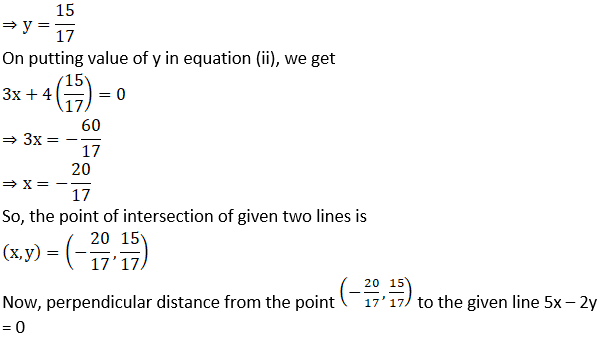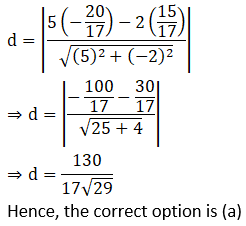# NCERT Exemplar Solutions for Class 11 Maths Chapter 10 Straight Lines

NCERT Exemplar Solutions for Class 11 Maths Chapter 10 Straight Lines are provided here, in downloadable PDF. These solutions are based on the latest CBSE syllabus and guidelines so that students can find it helpful to solve the exercise questions present in the textbooks. These solutions are prepared by a set of subject-matter experts at BYJU’S to clear the doubts of students. We have covered all the questions with which the question papers could be prepared for the final exams. Therefore, these NCERT Exemplar Solutions are very helpful for students to use as a reference tool while preparing for the annual examinations.

Students can effectively start practising NCERT Exemplar Solutions for Class 11, which will build a strong grip on Maths and will result in better performance in the annual exam.  NCERT Exemplar Solutions for Class 11 Maths Chapter 10, Straight Lines, explains the concepts related to straight lines. Some of the crucial topics of this chapter are listed below:

• The slope of a line
• The angle between two lines
• Collinearity of three points
• Various forms of the equation of a line
• General equation of a line
• A distance of a point from a line
• Distance between two parallel lines
• Locus and equation of Locus
• The intersection of two given lines

## Download the PDF of NCERT Exemplar Solutions for Class 11 Maths Chapter 10 Straight Lines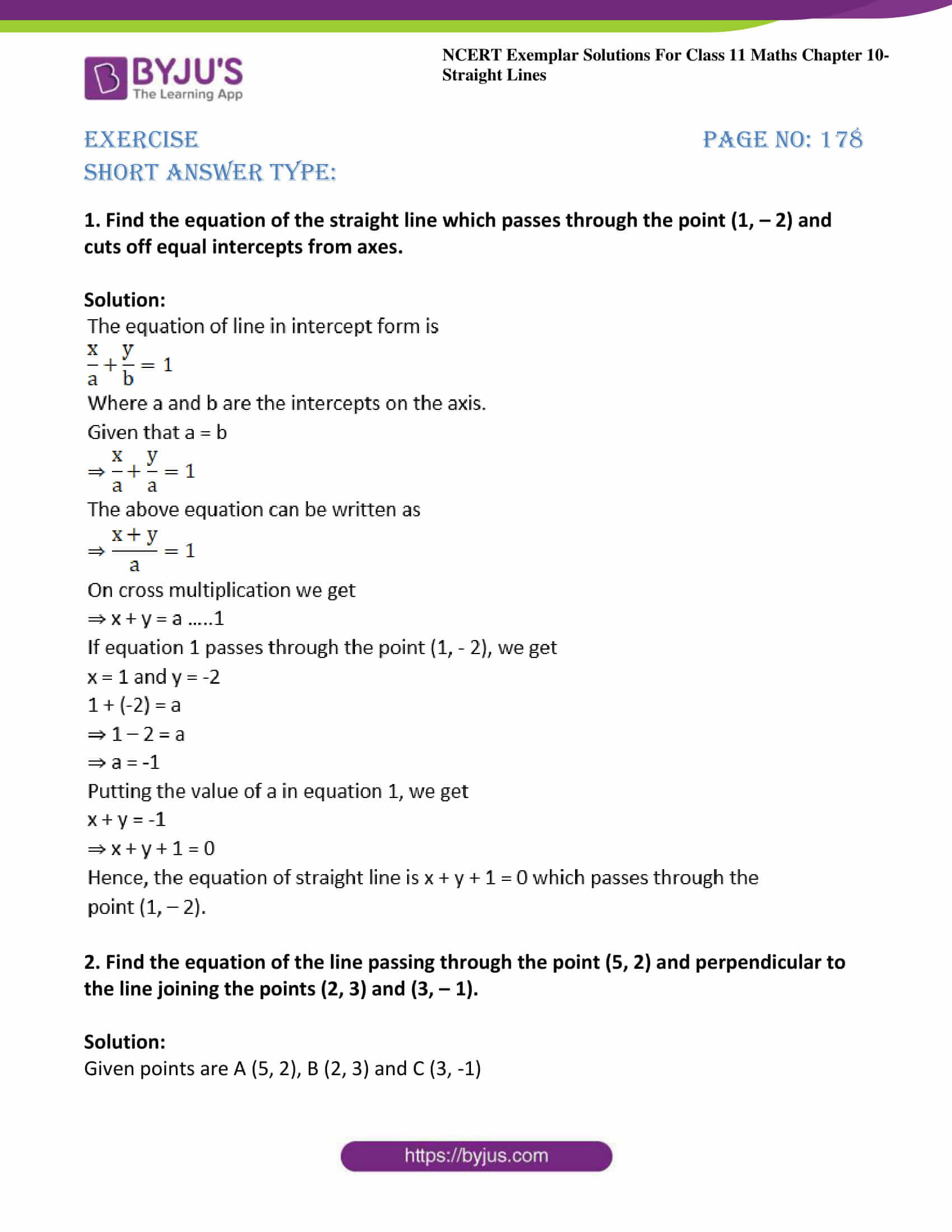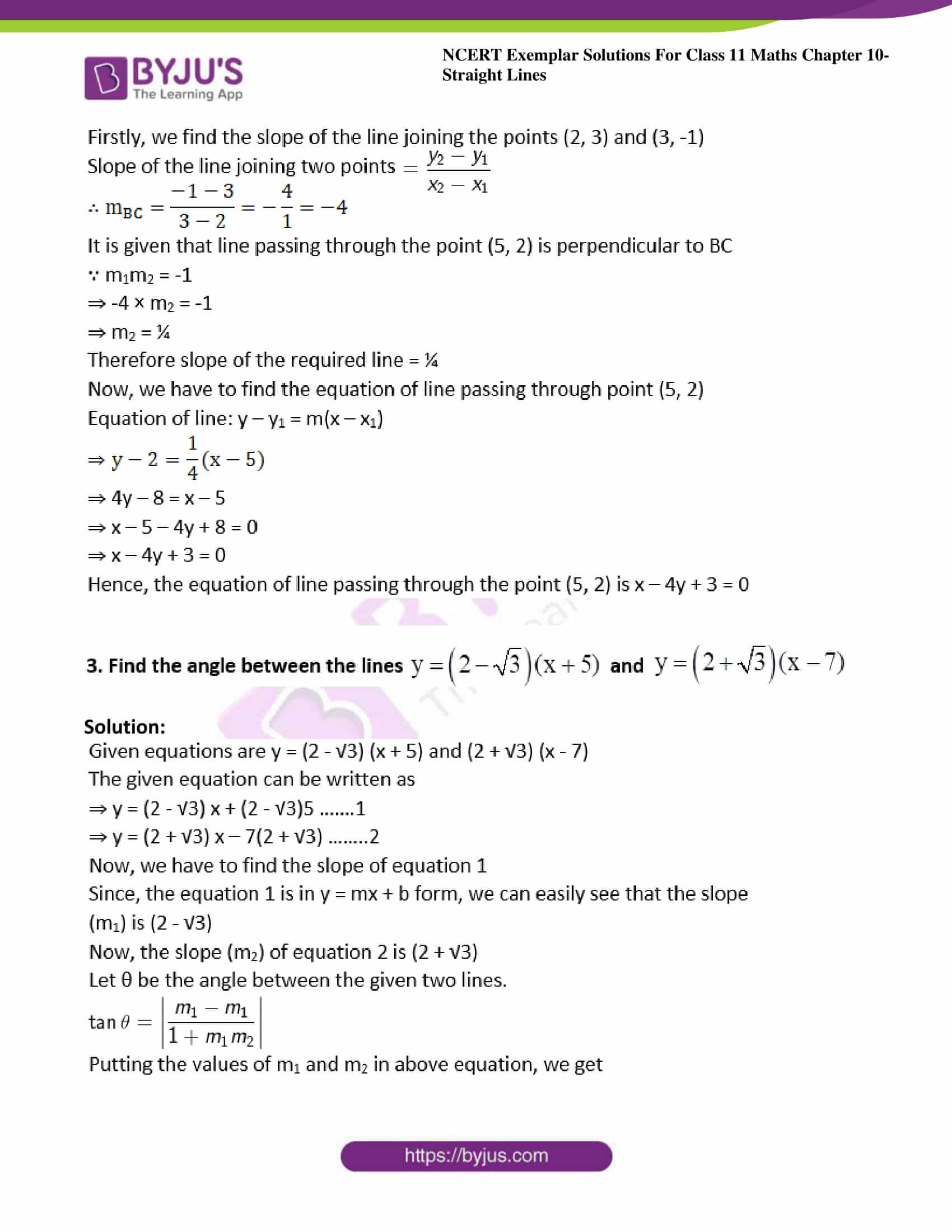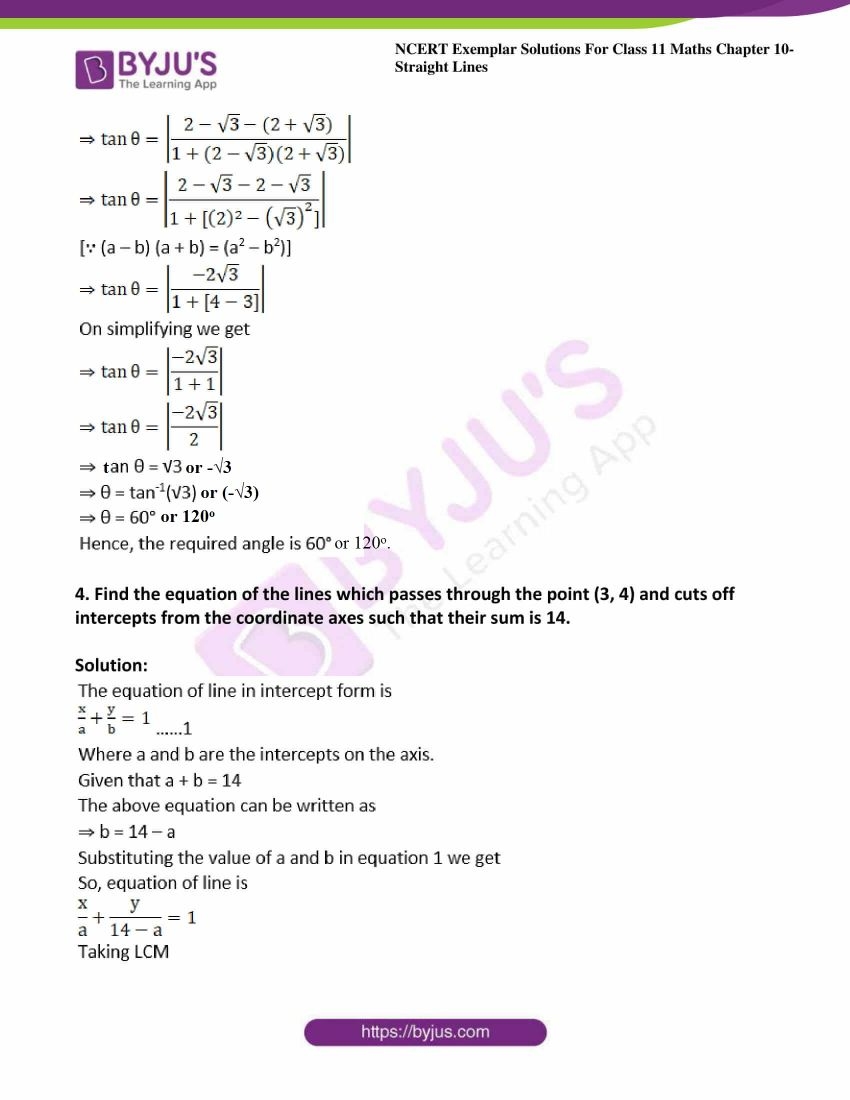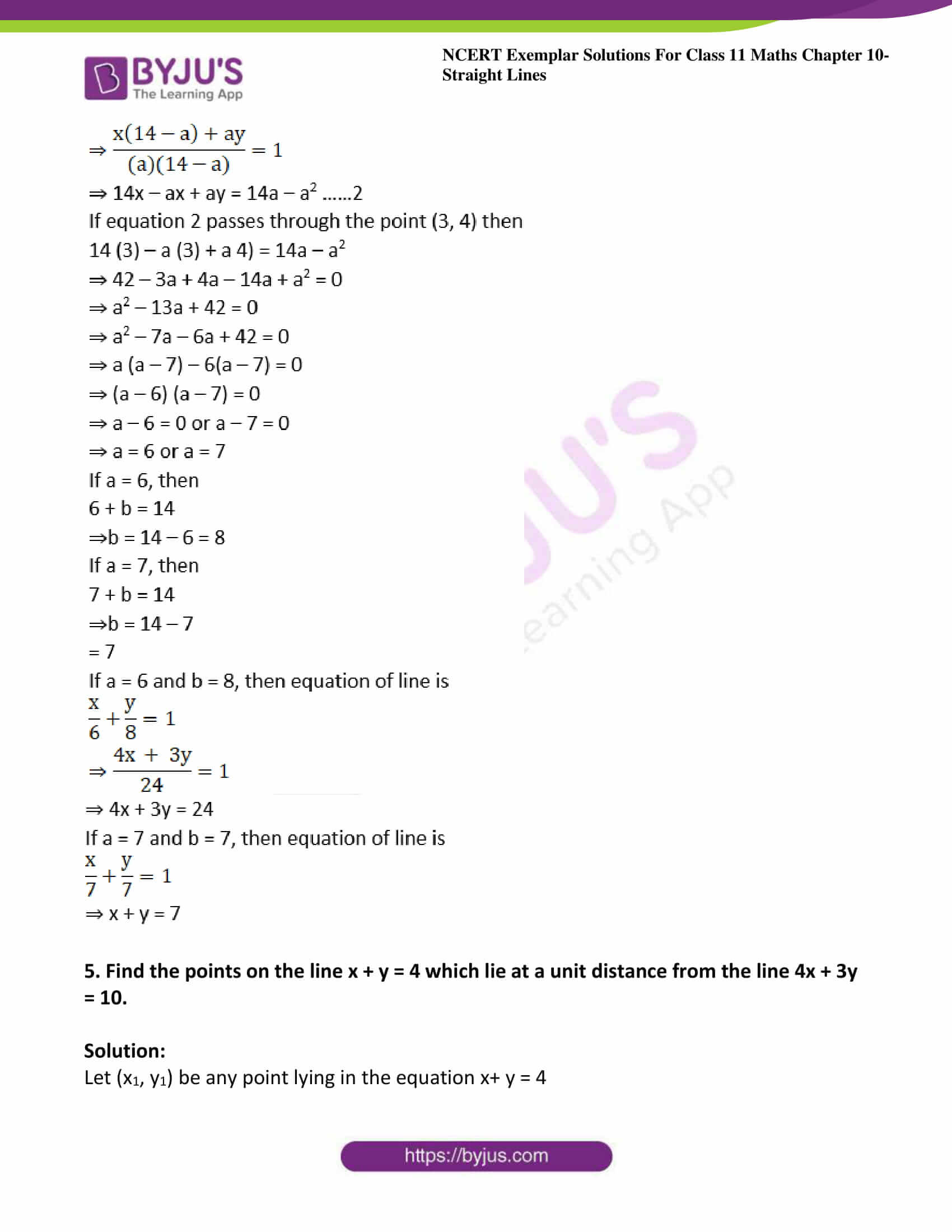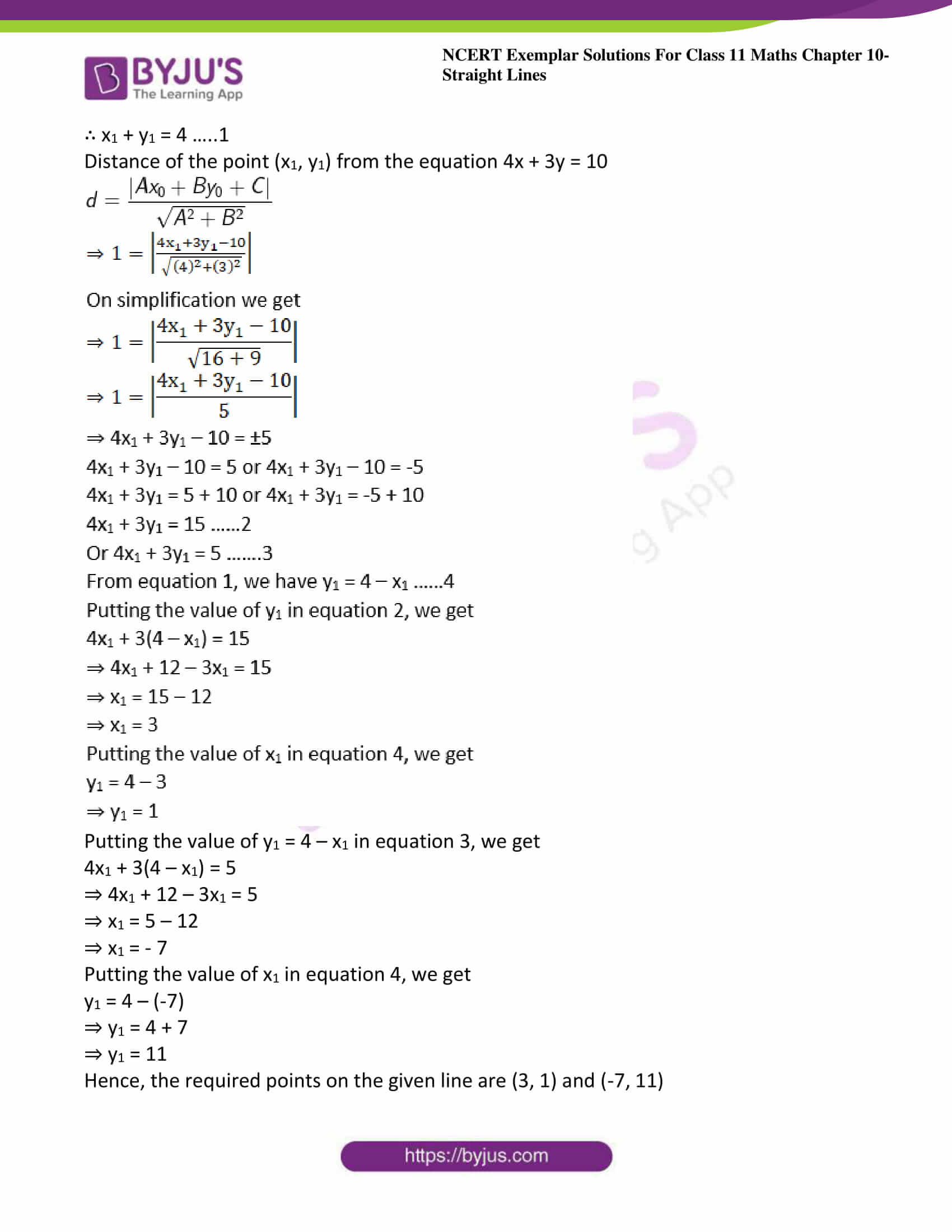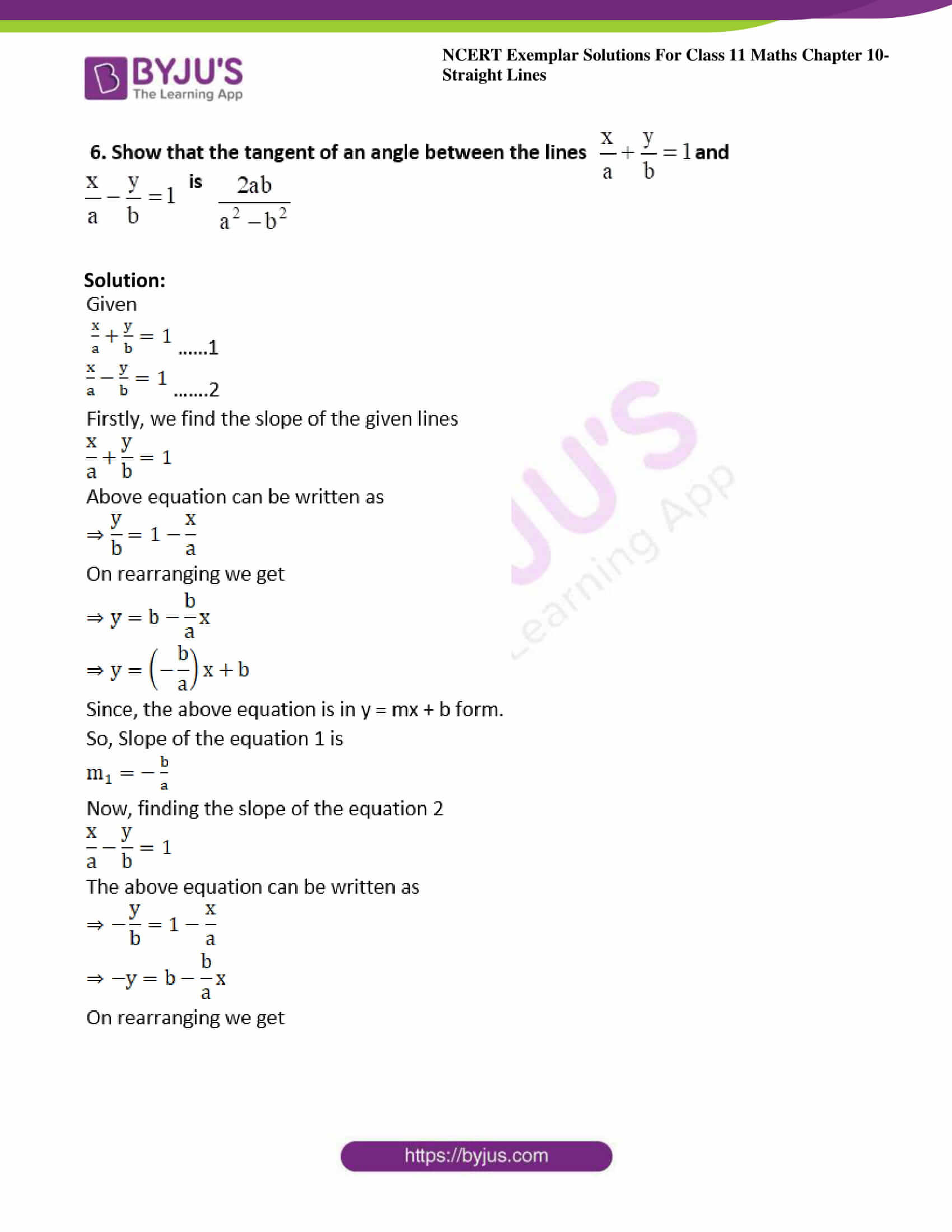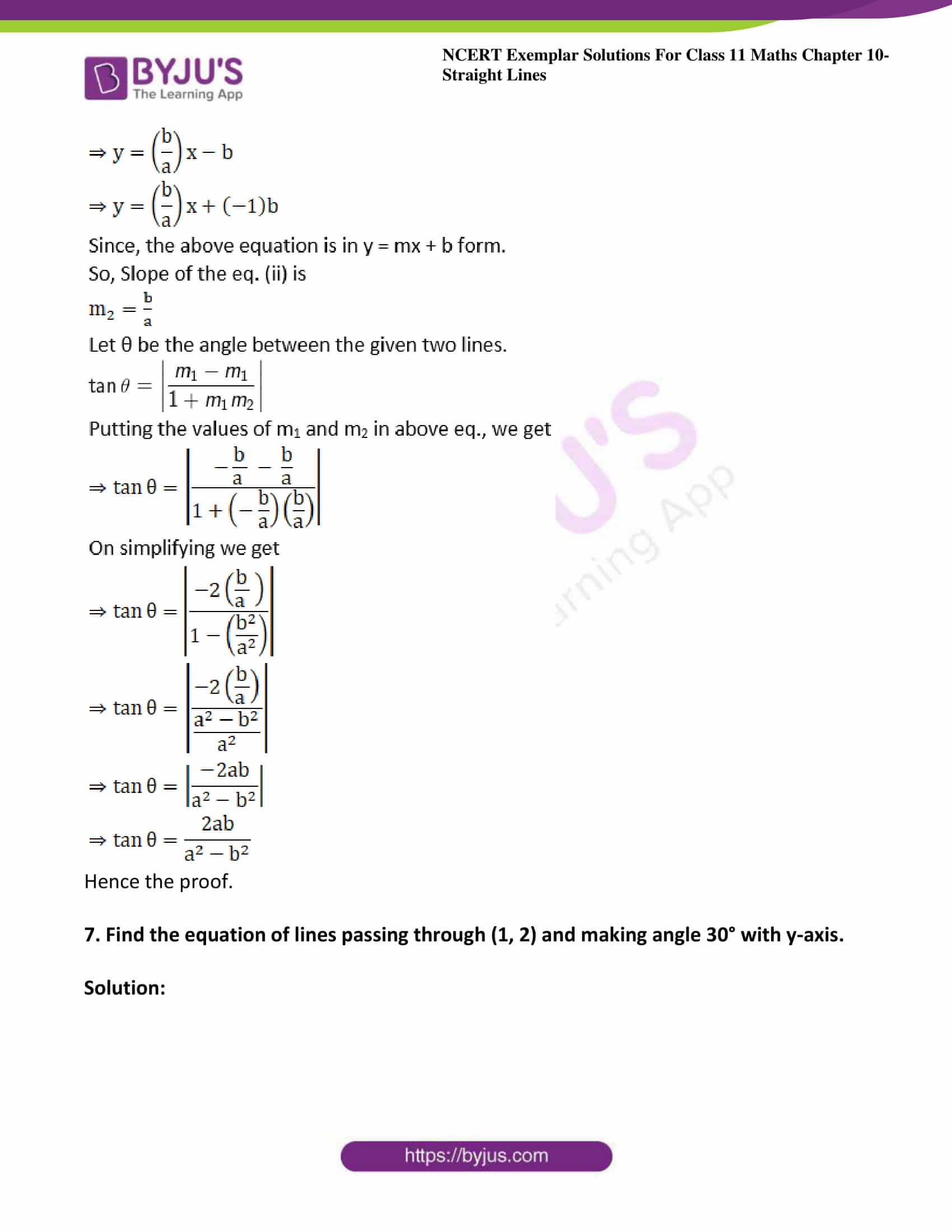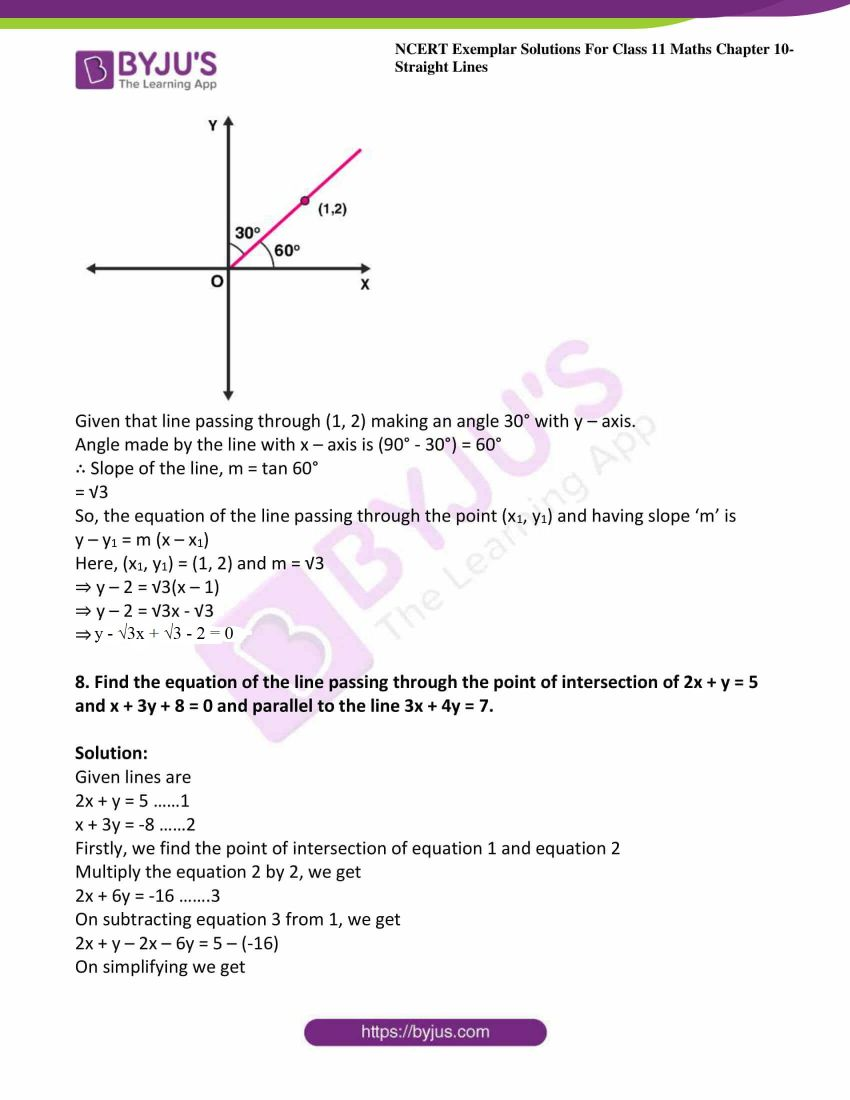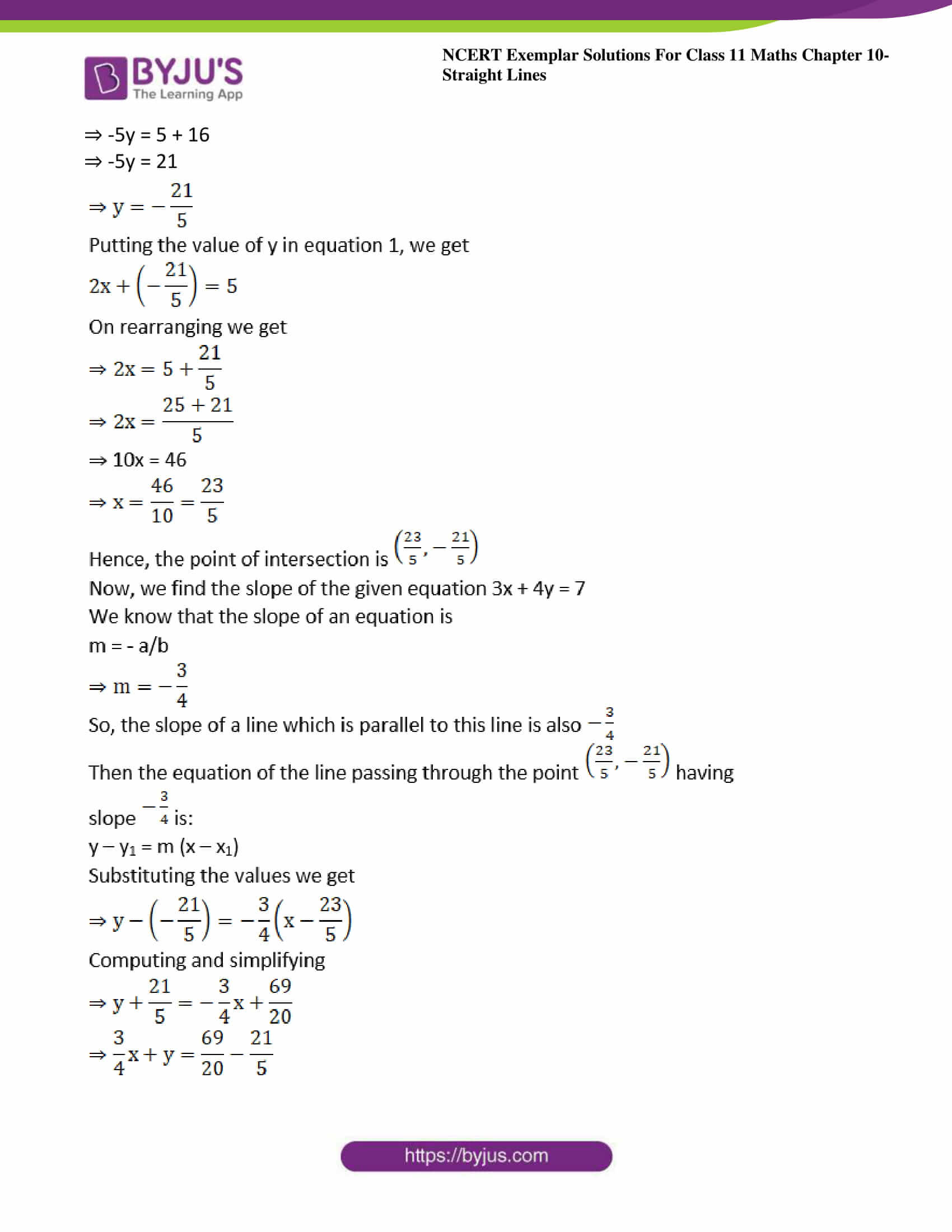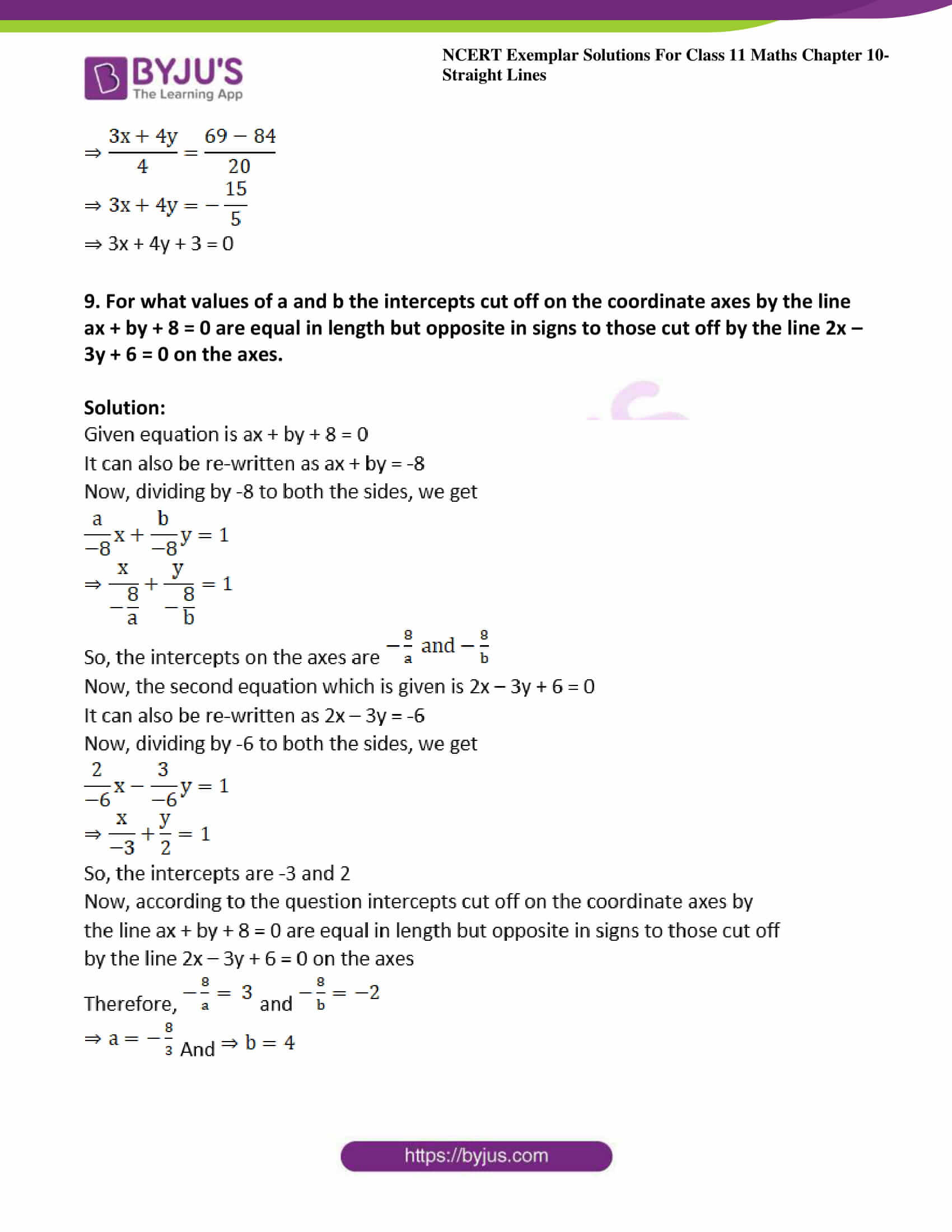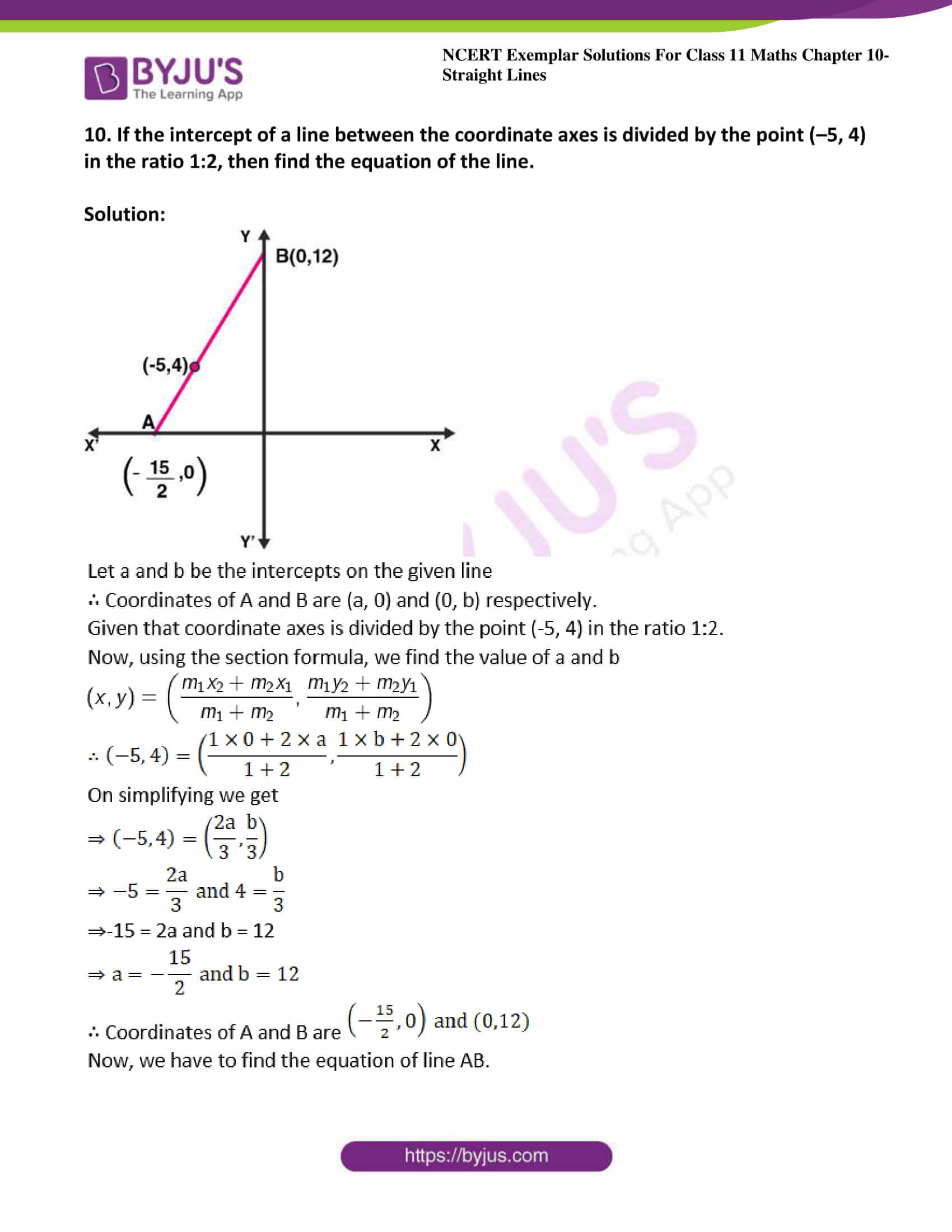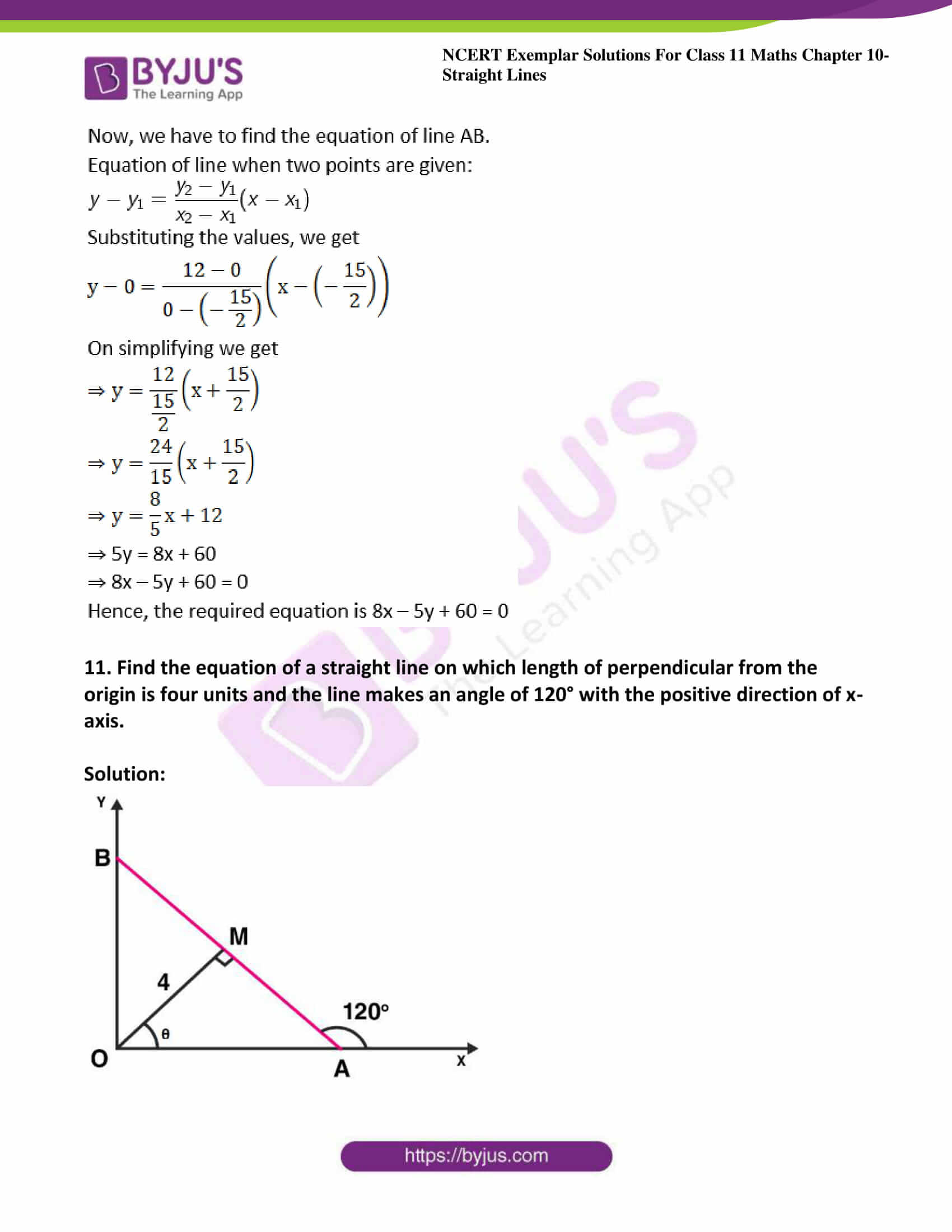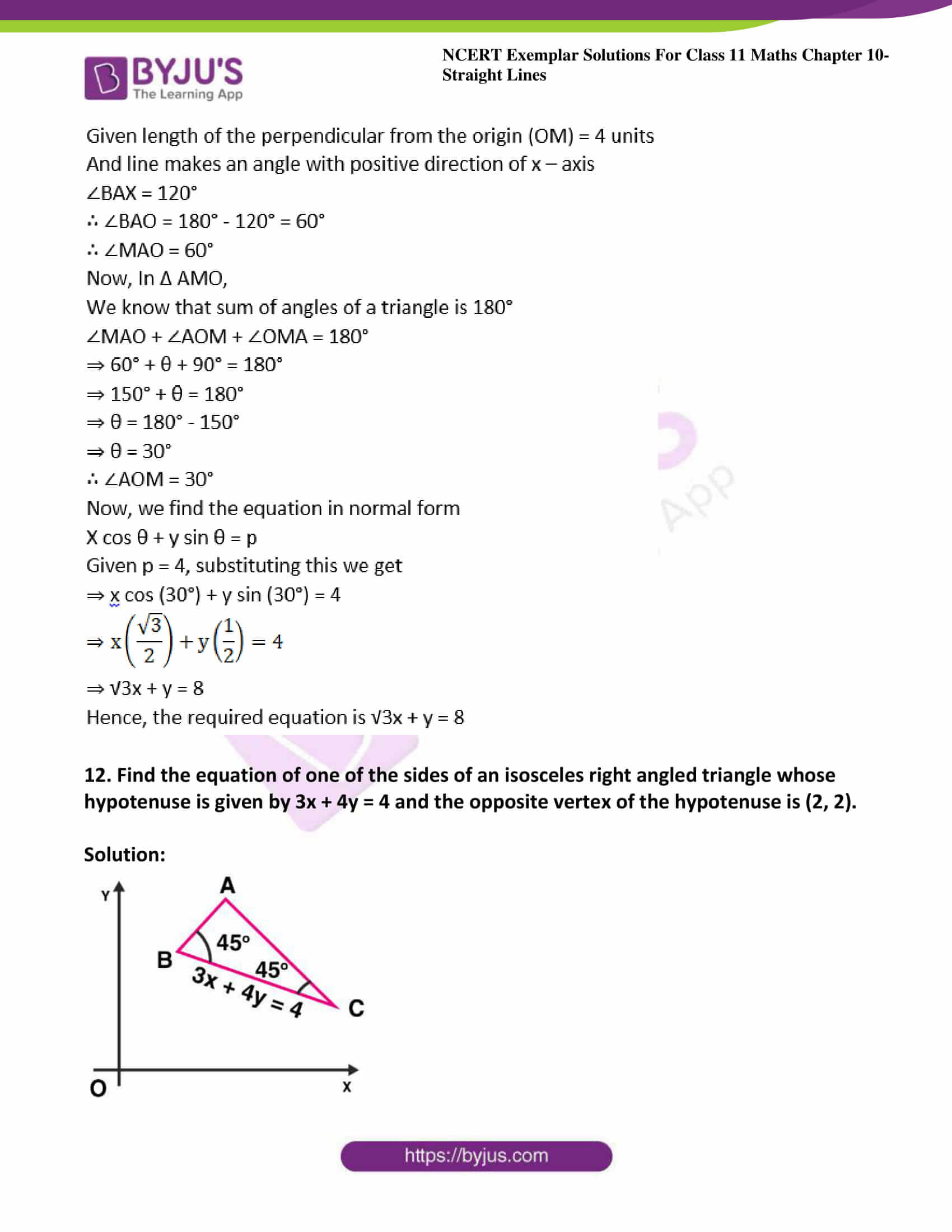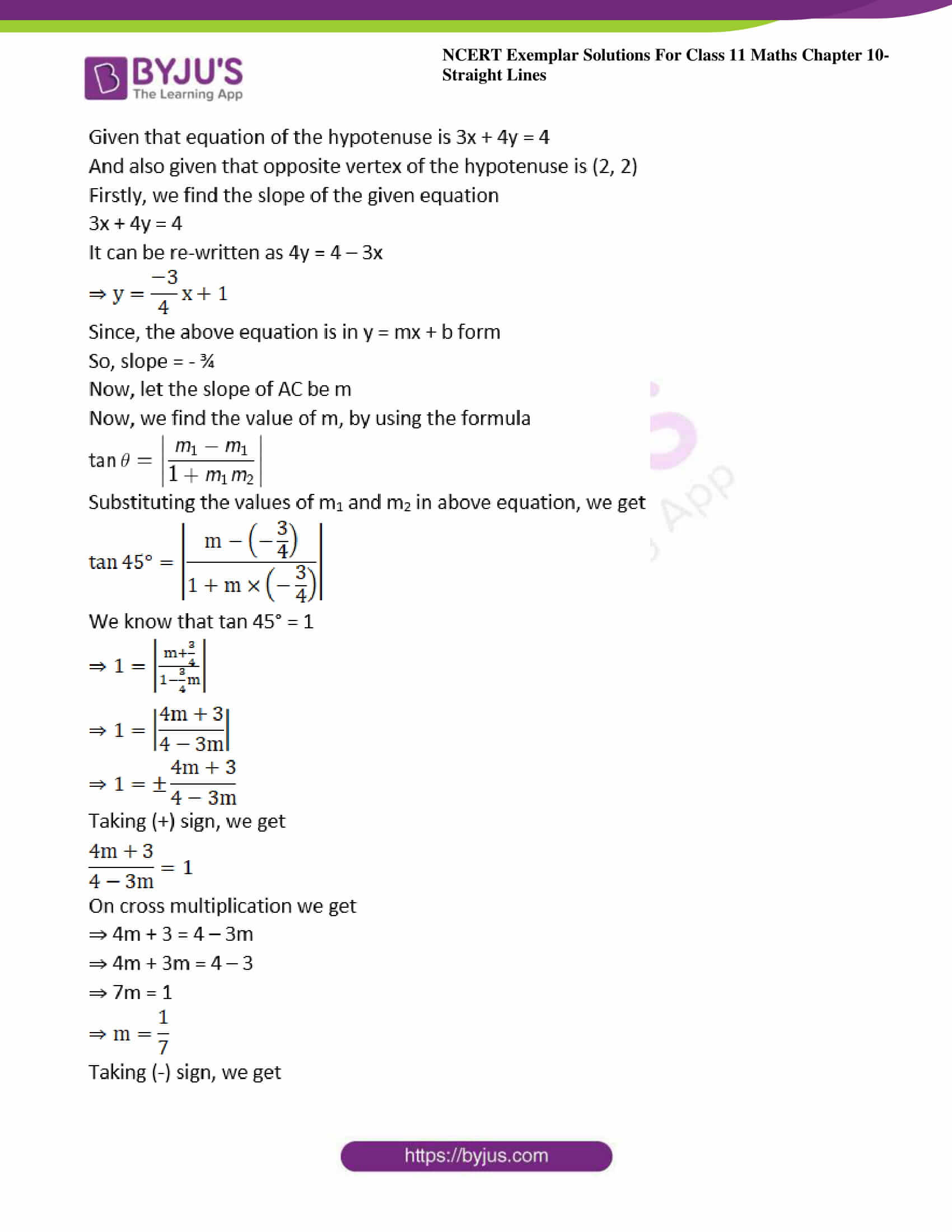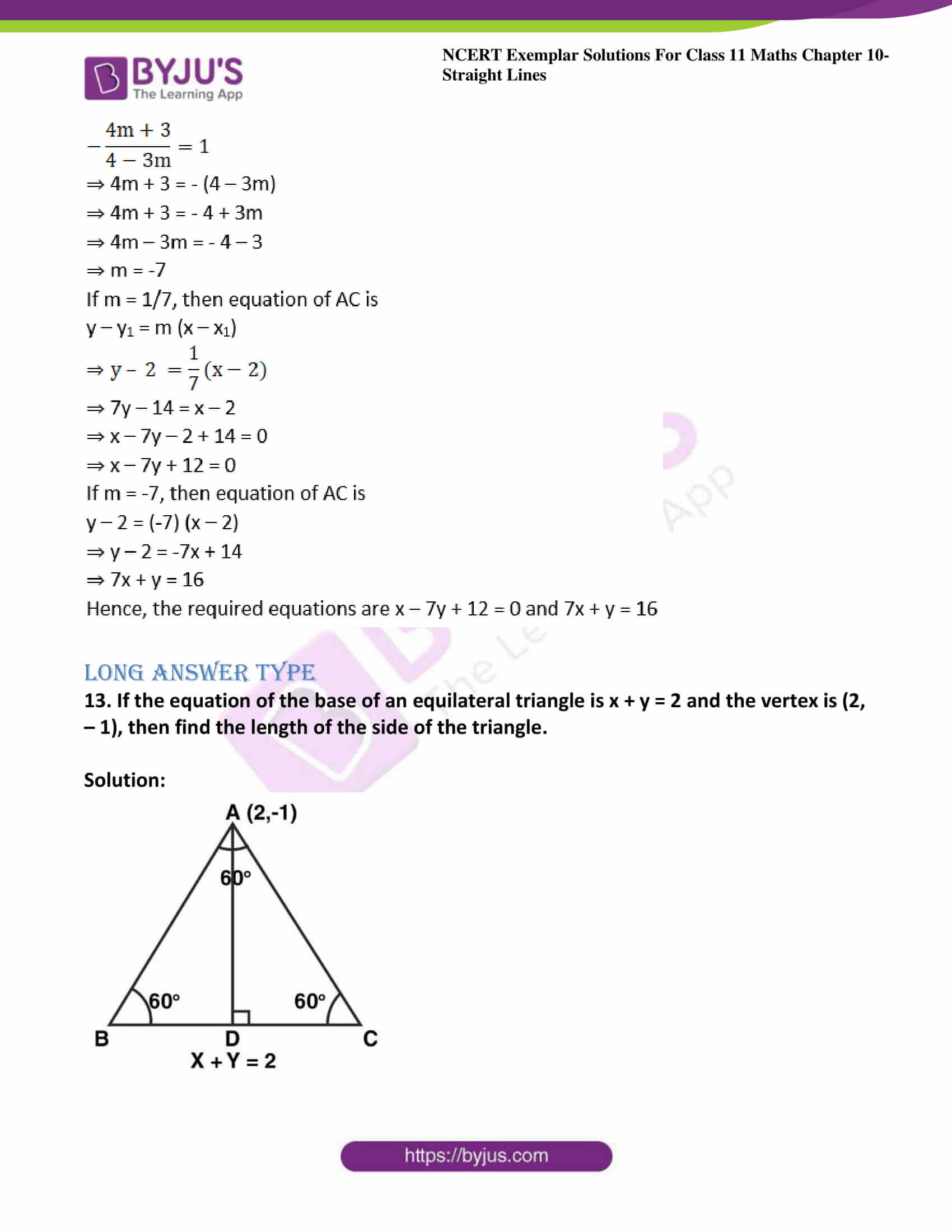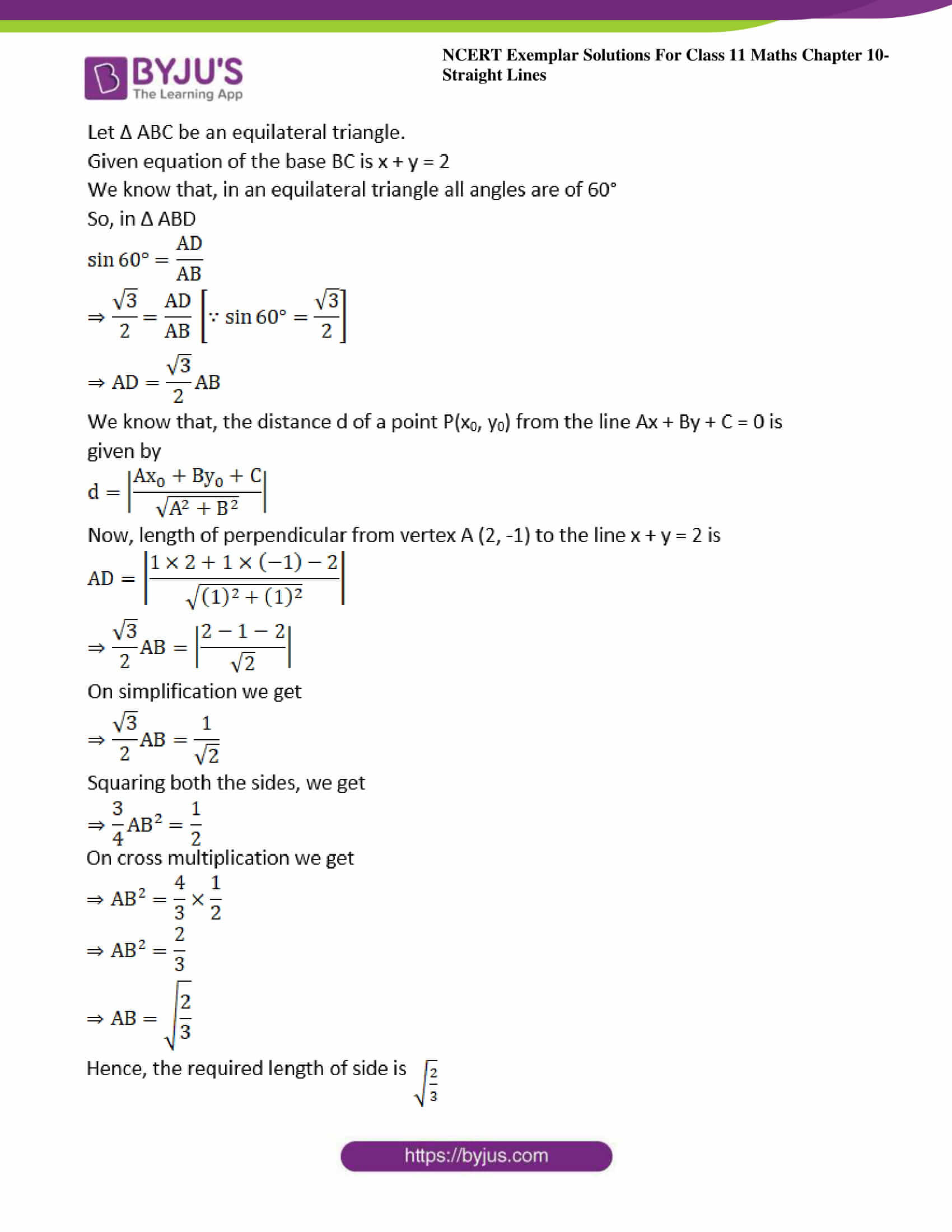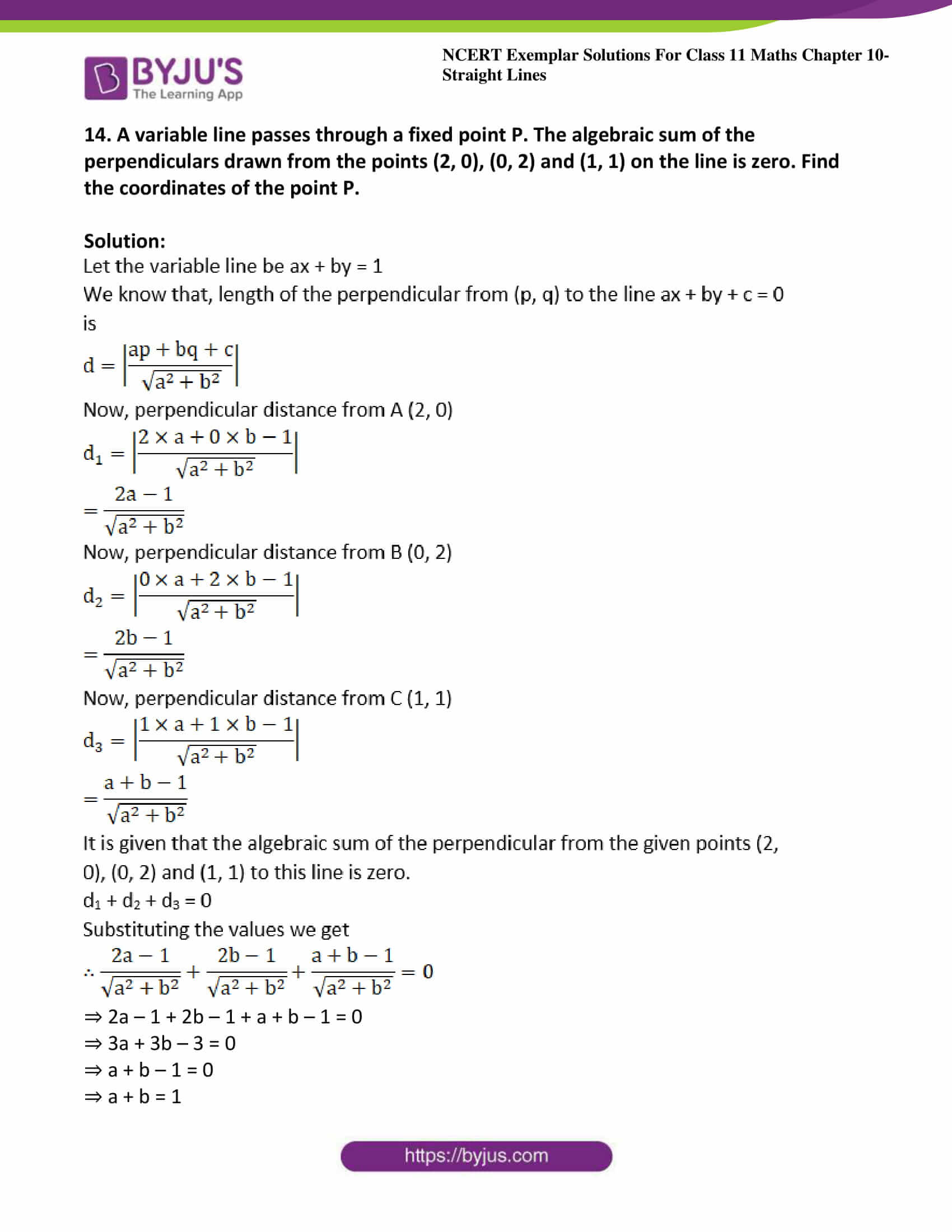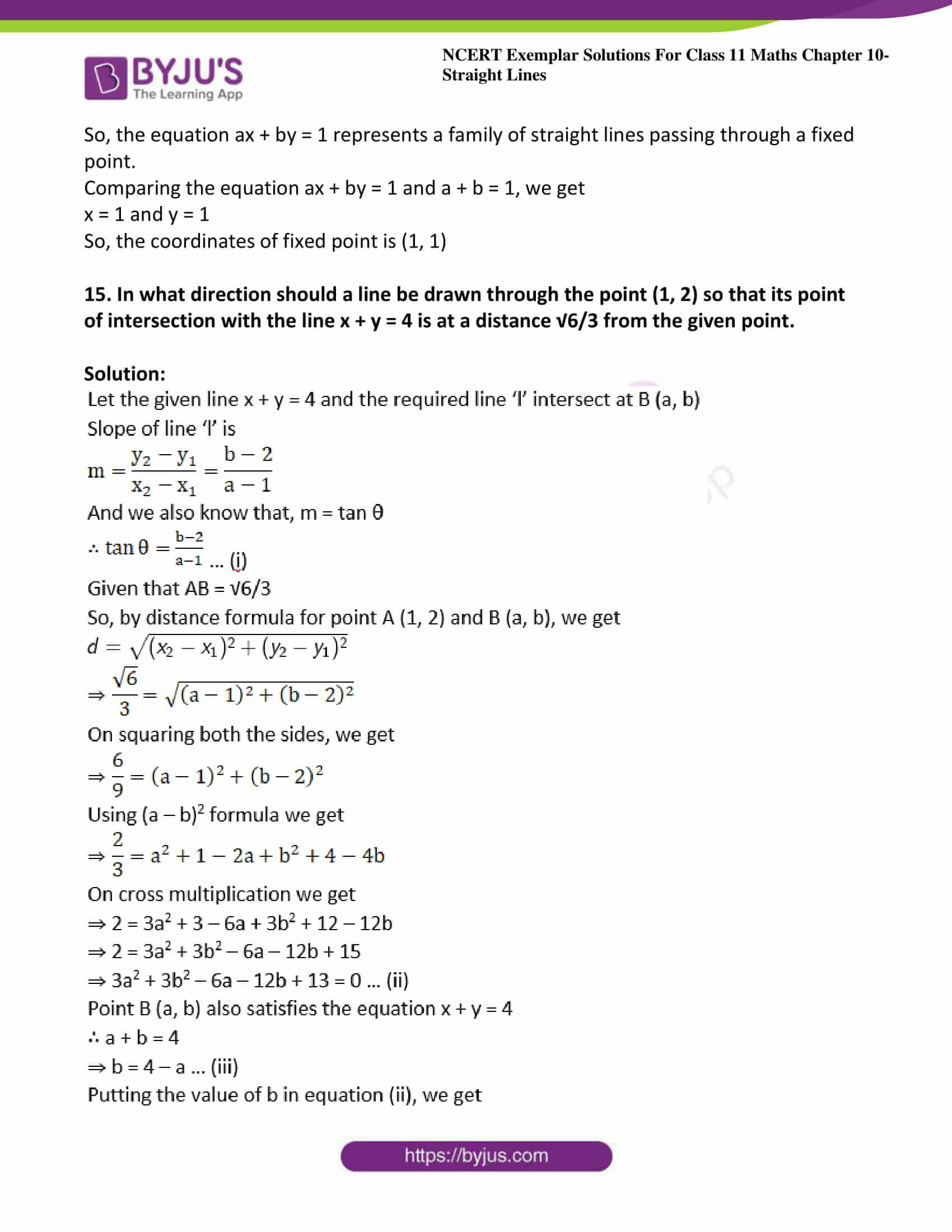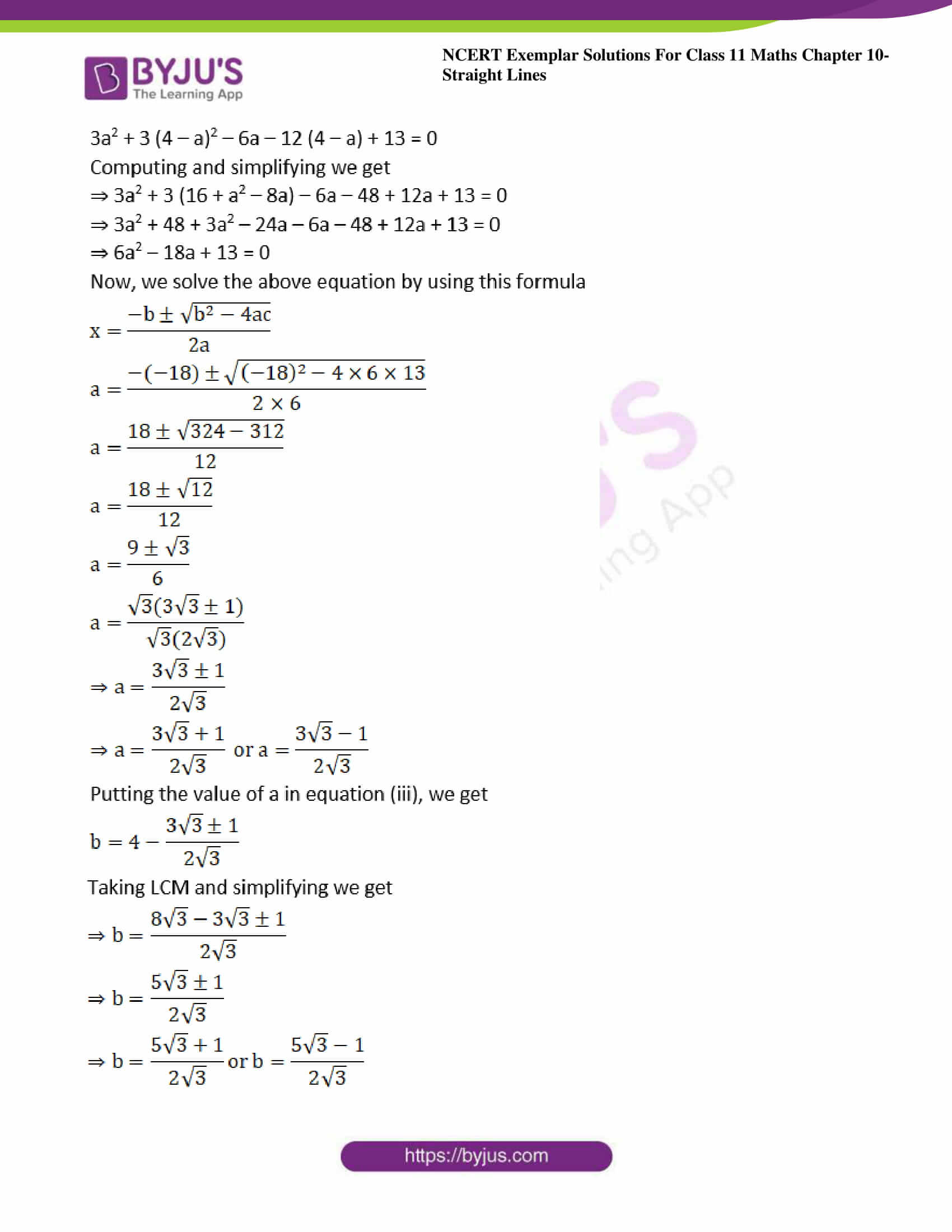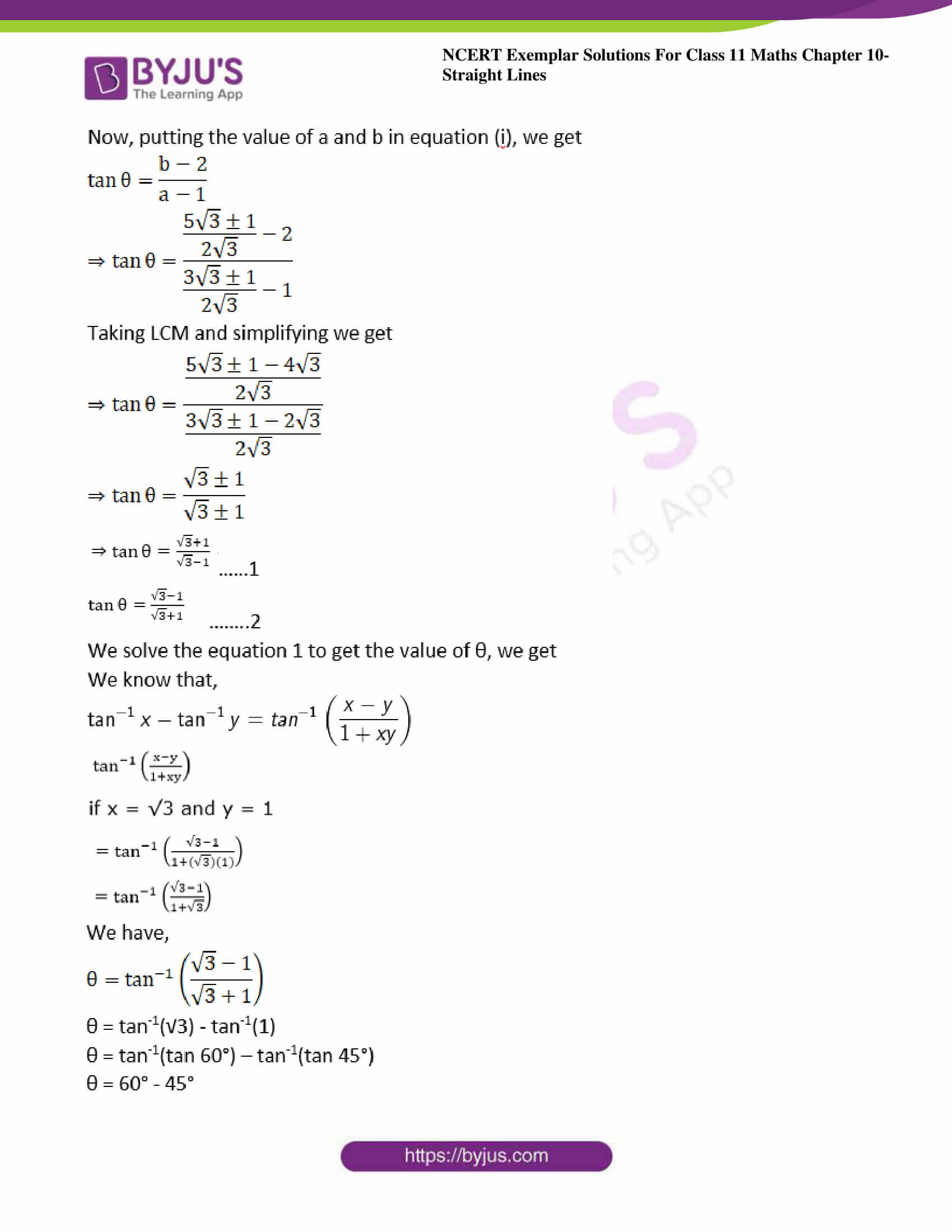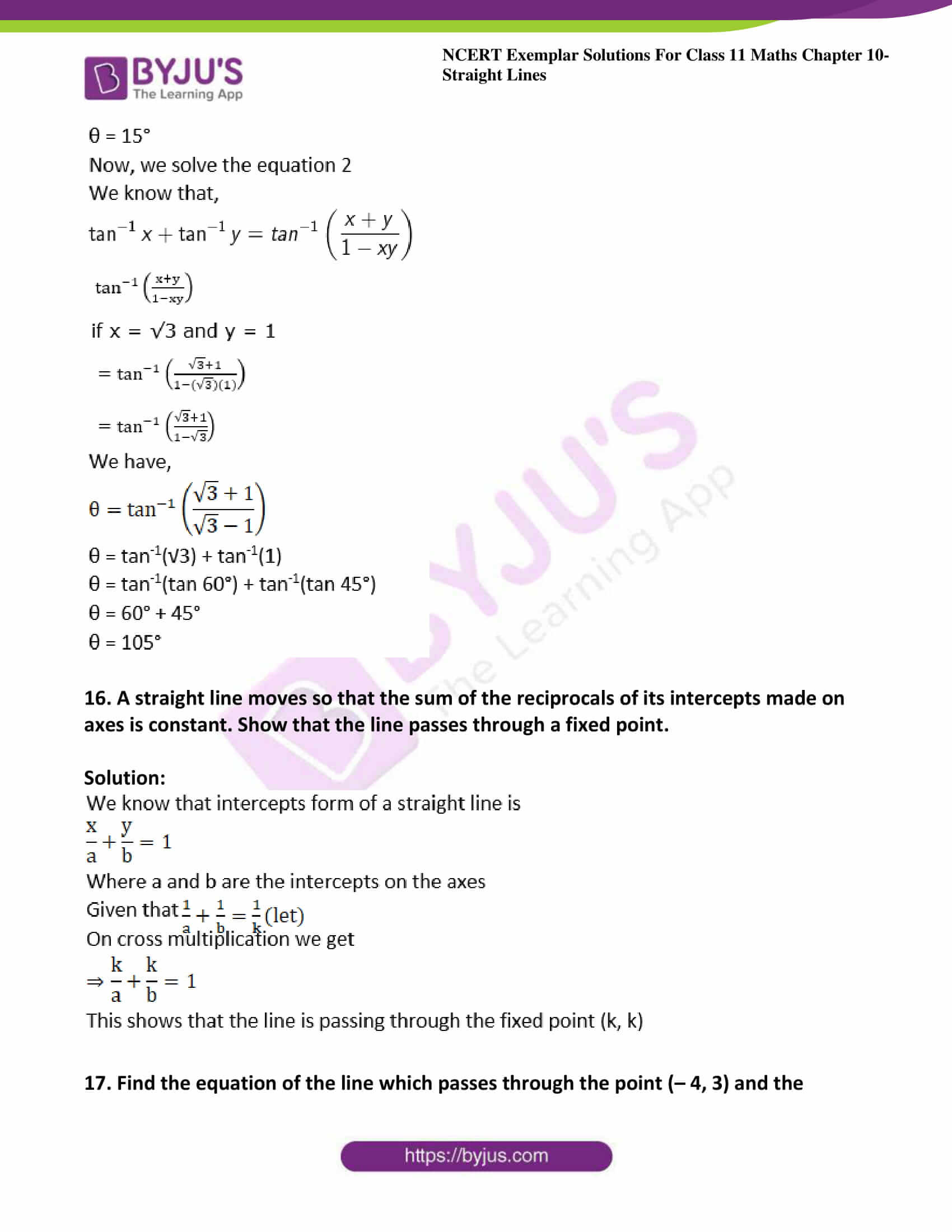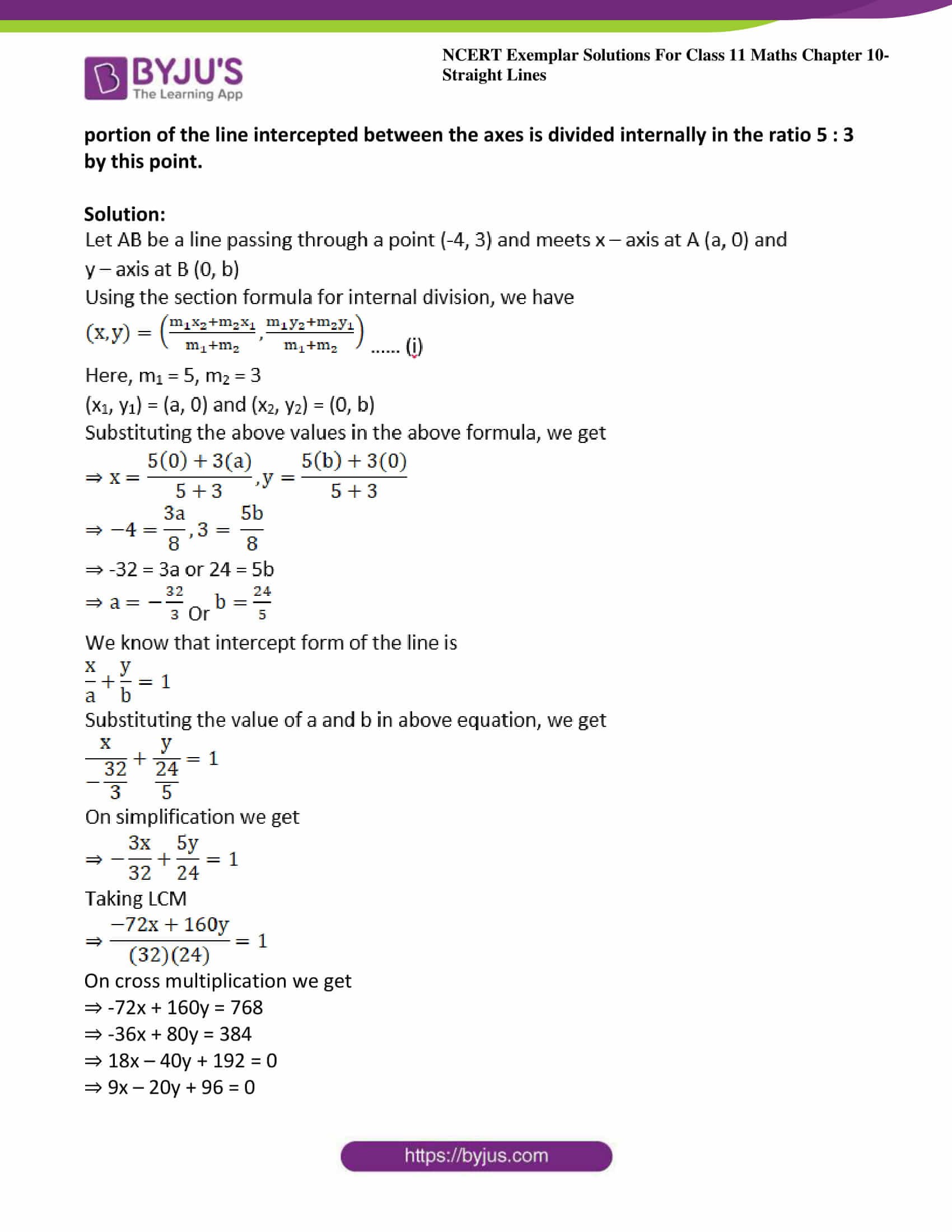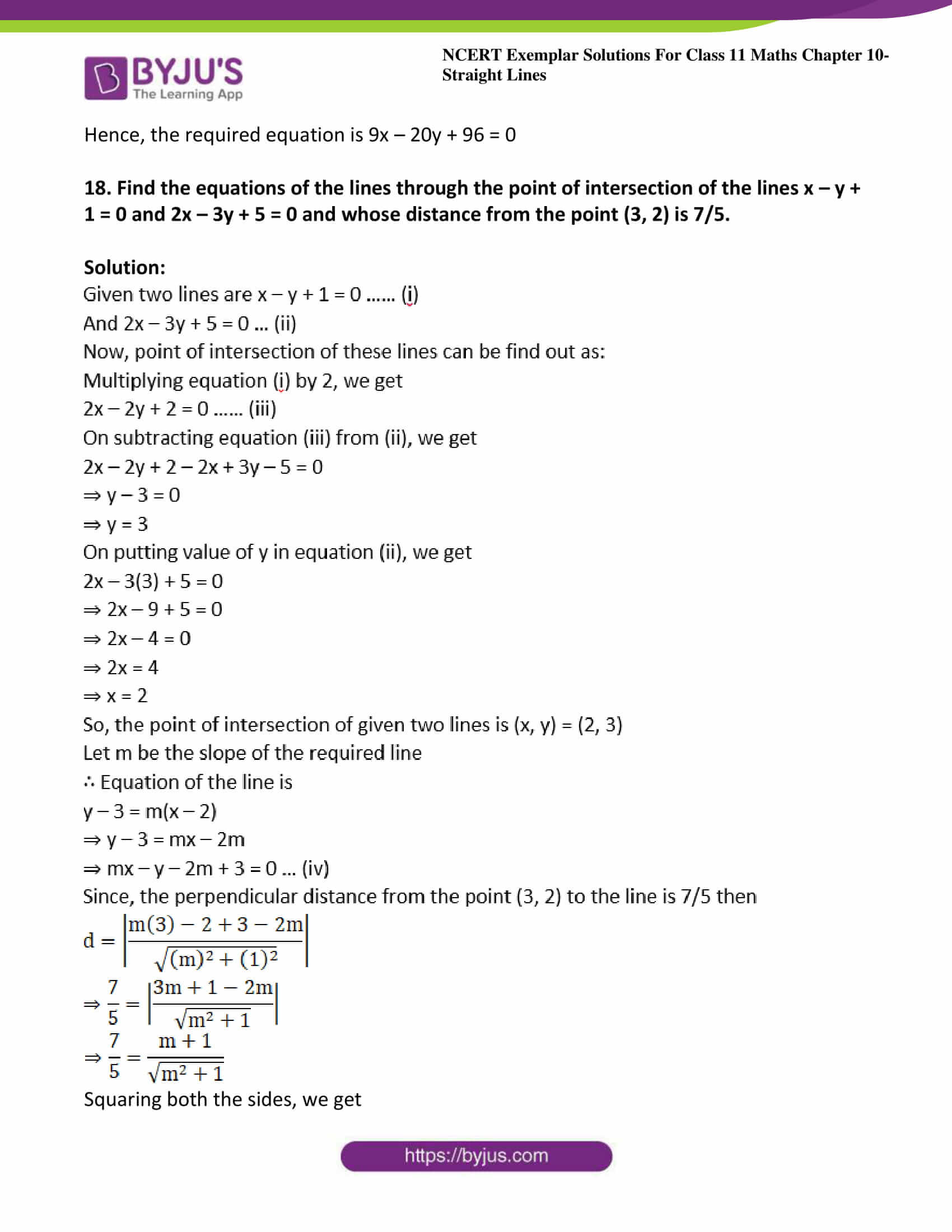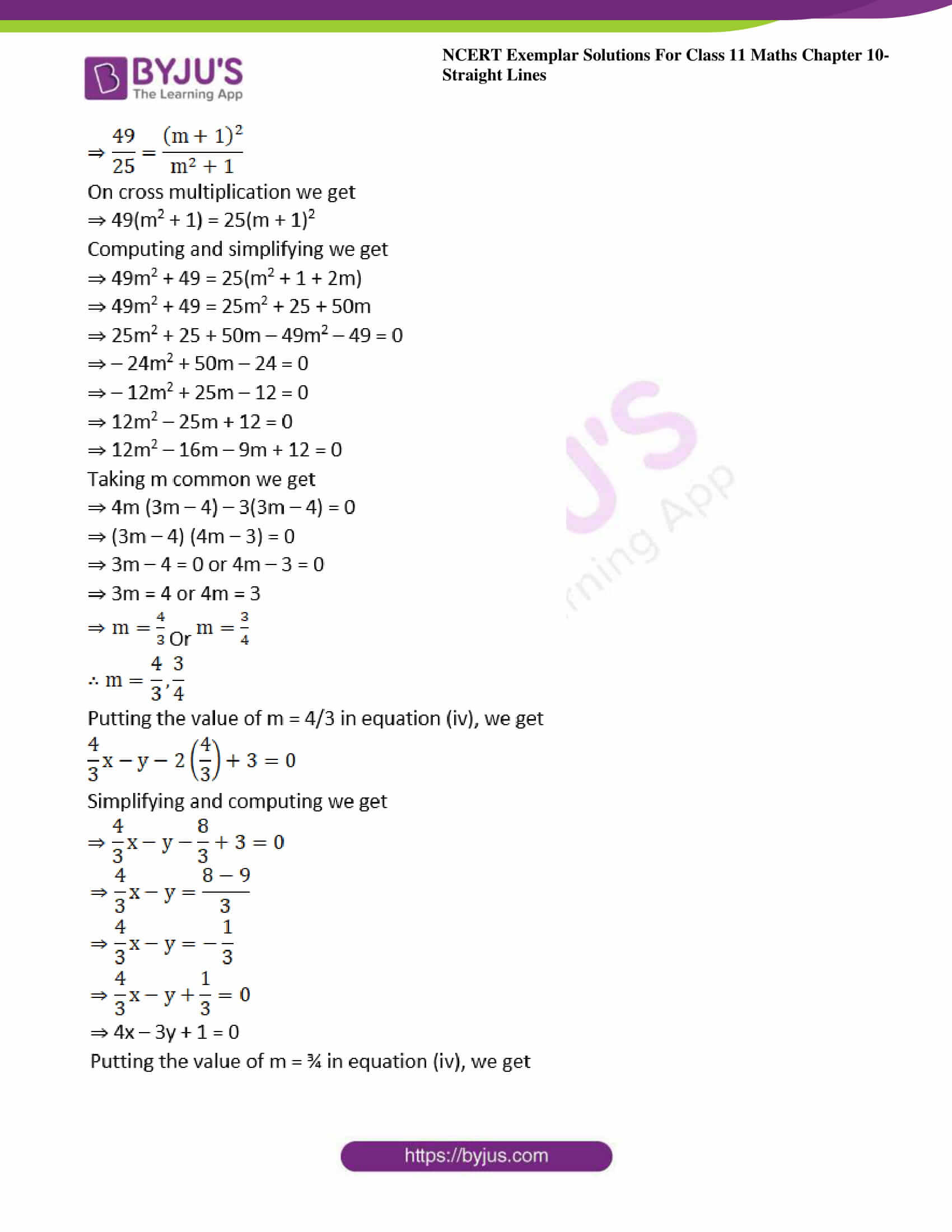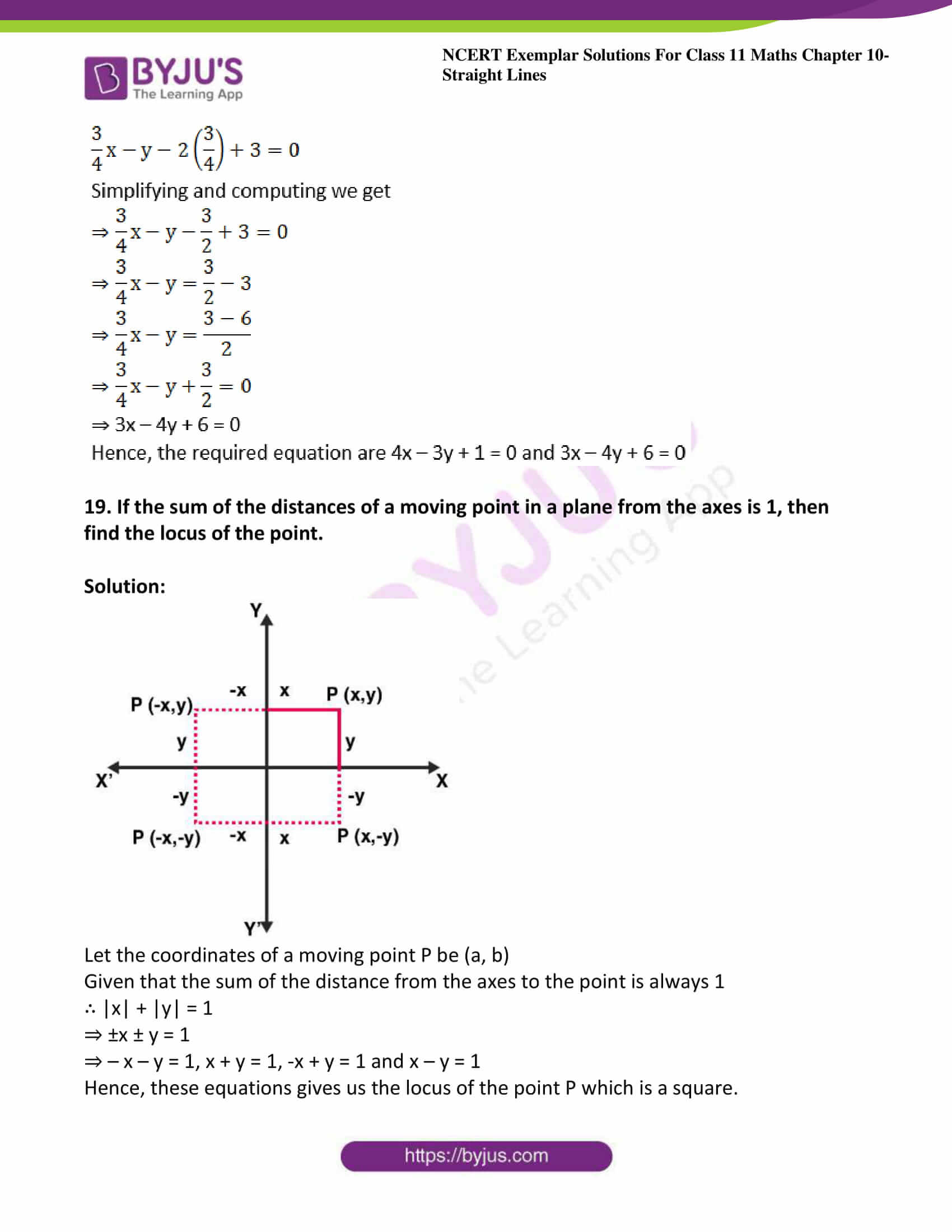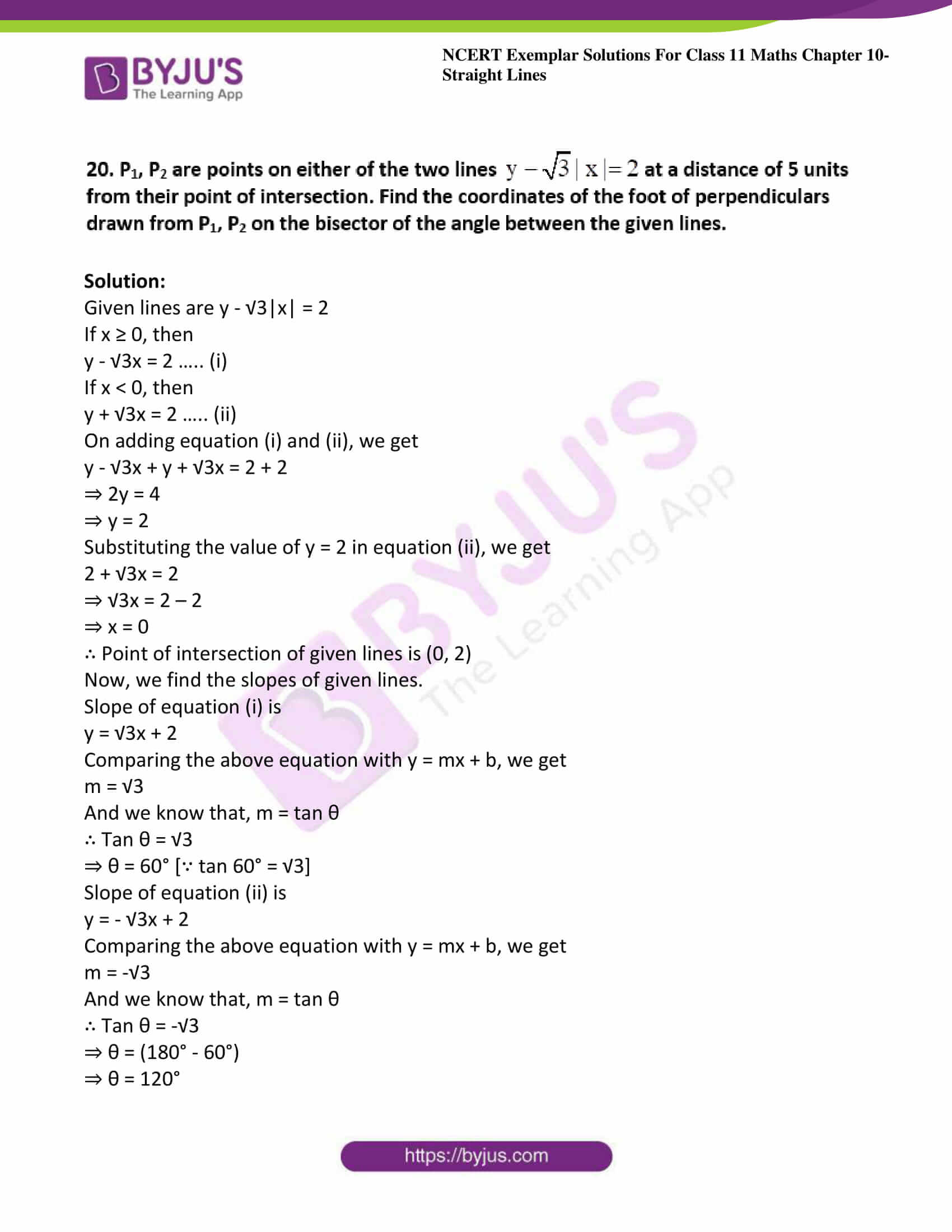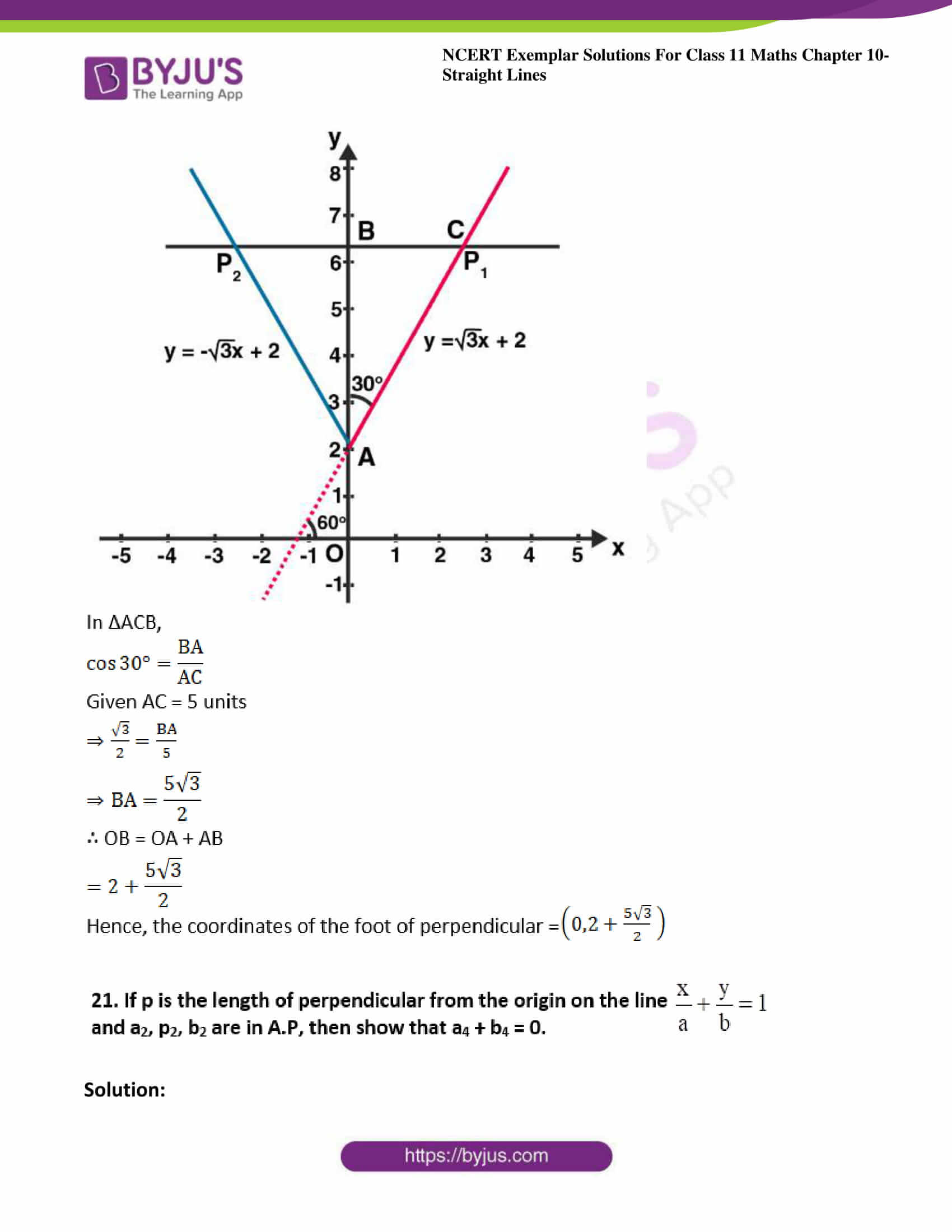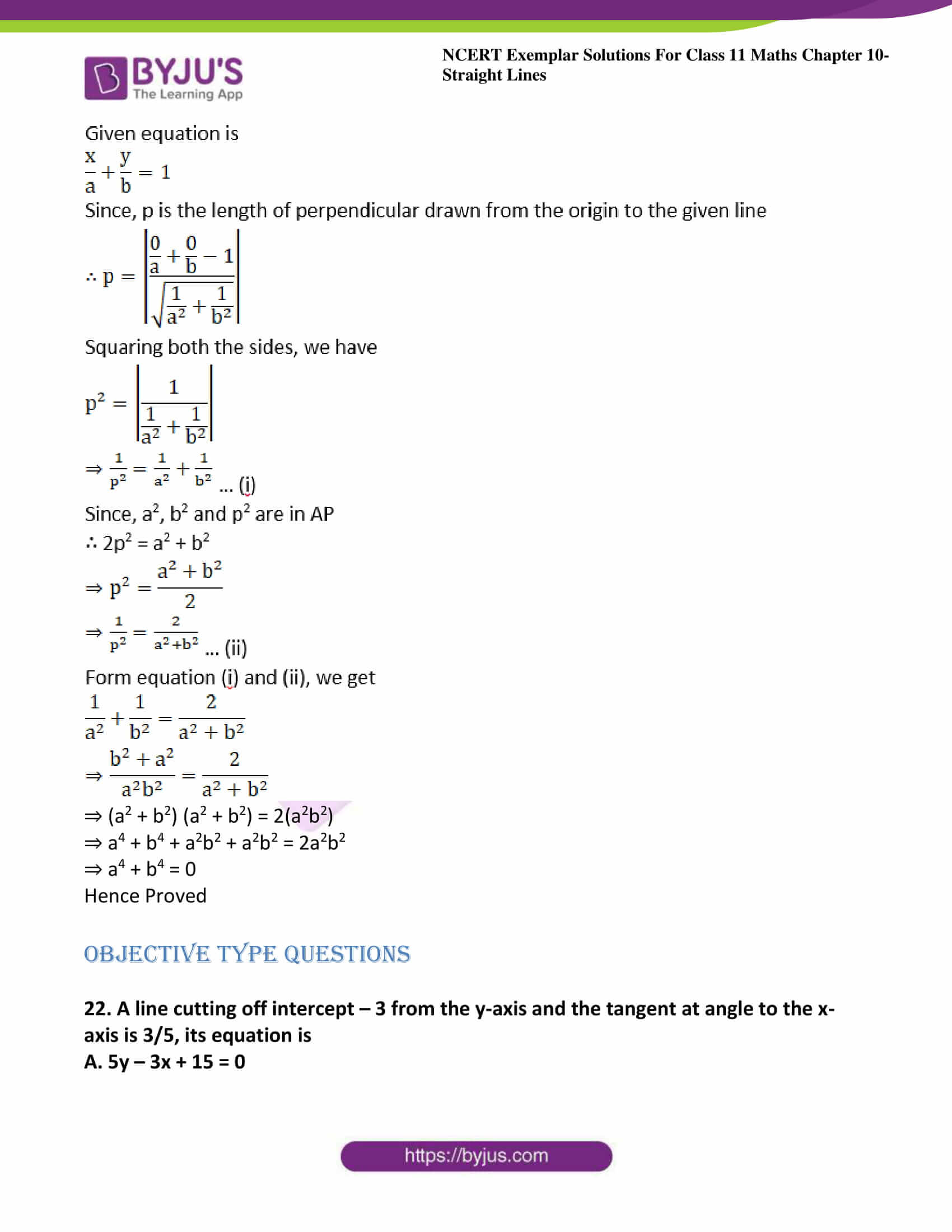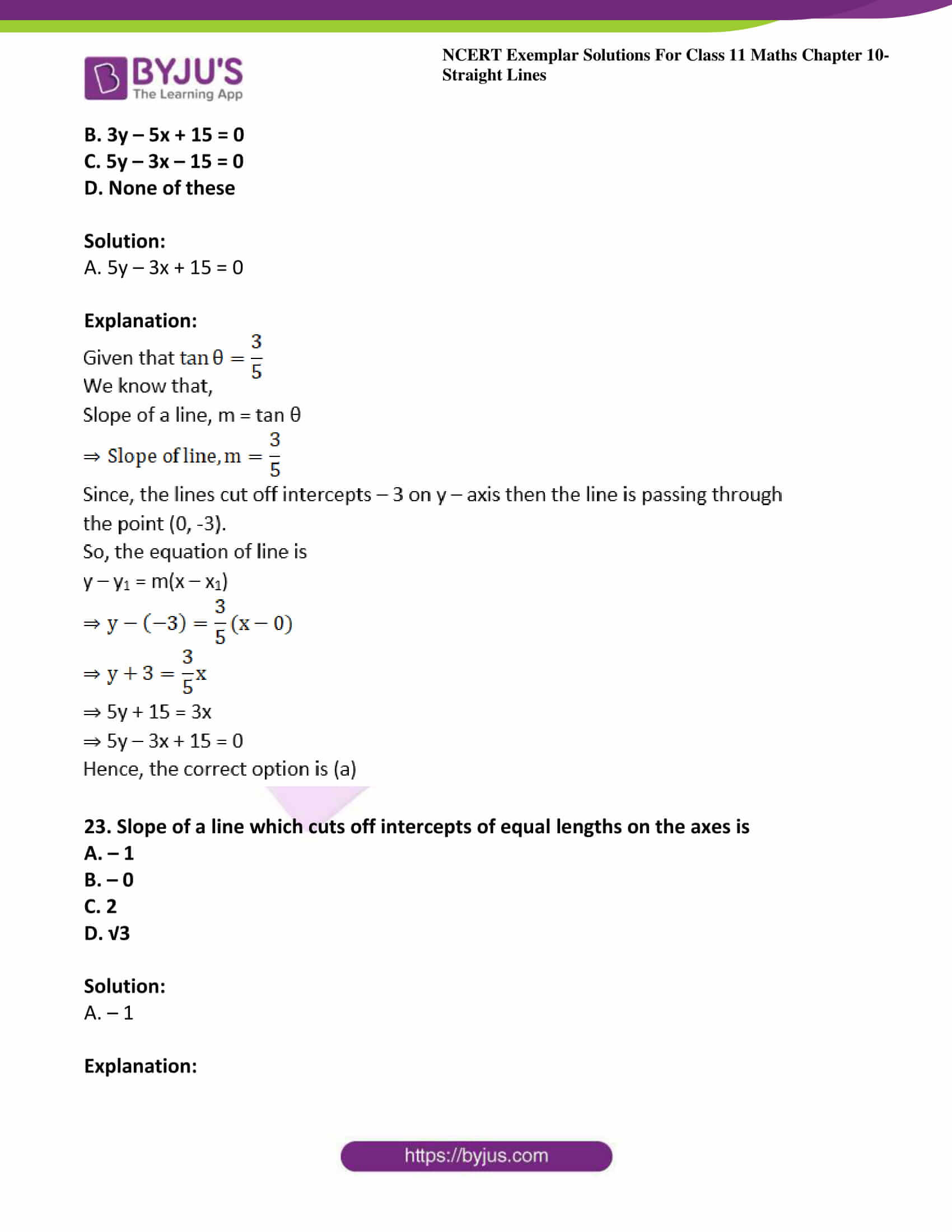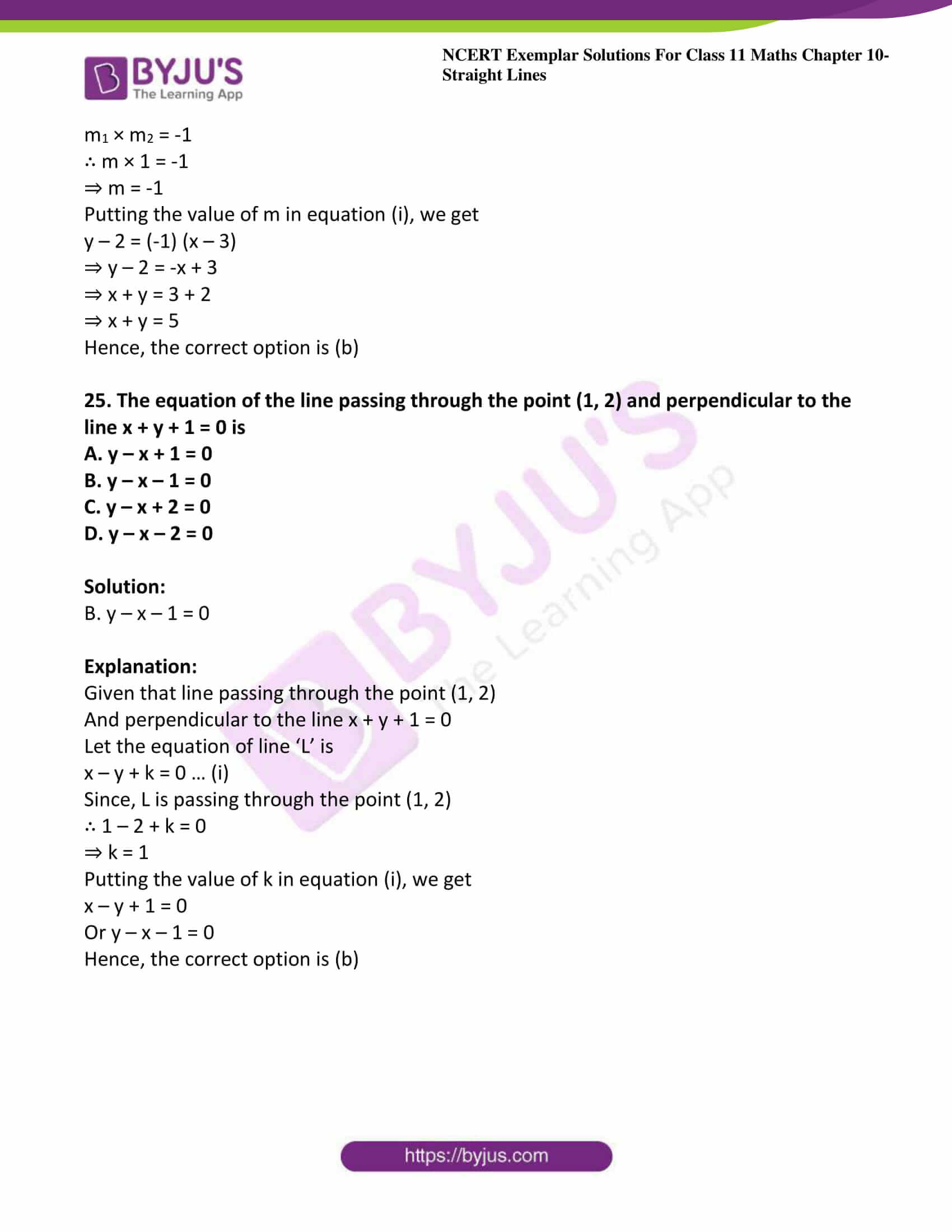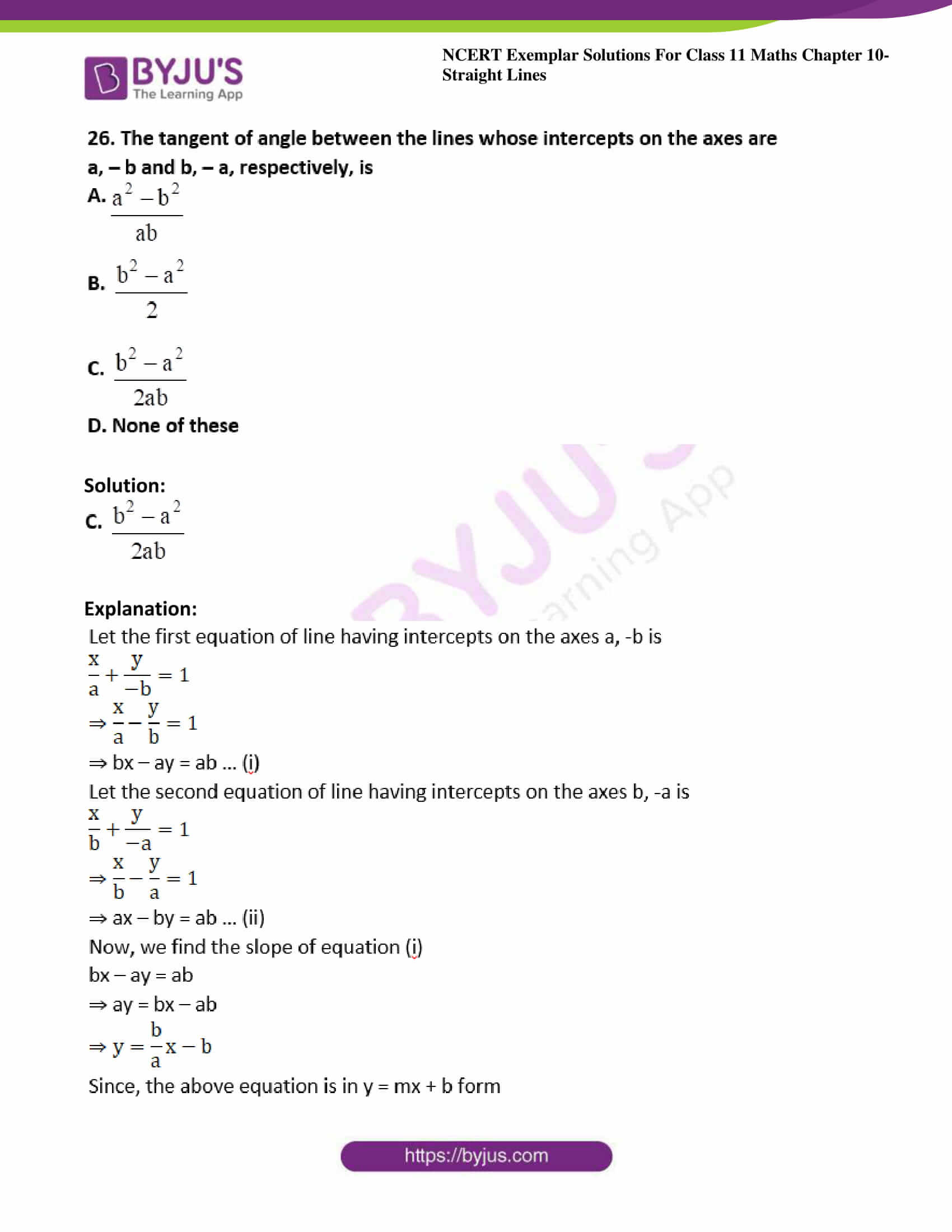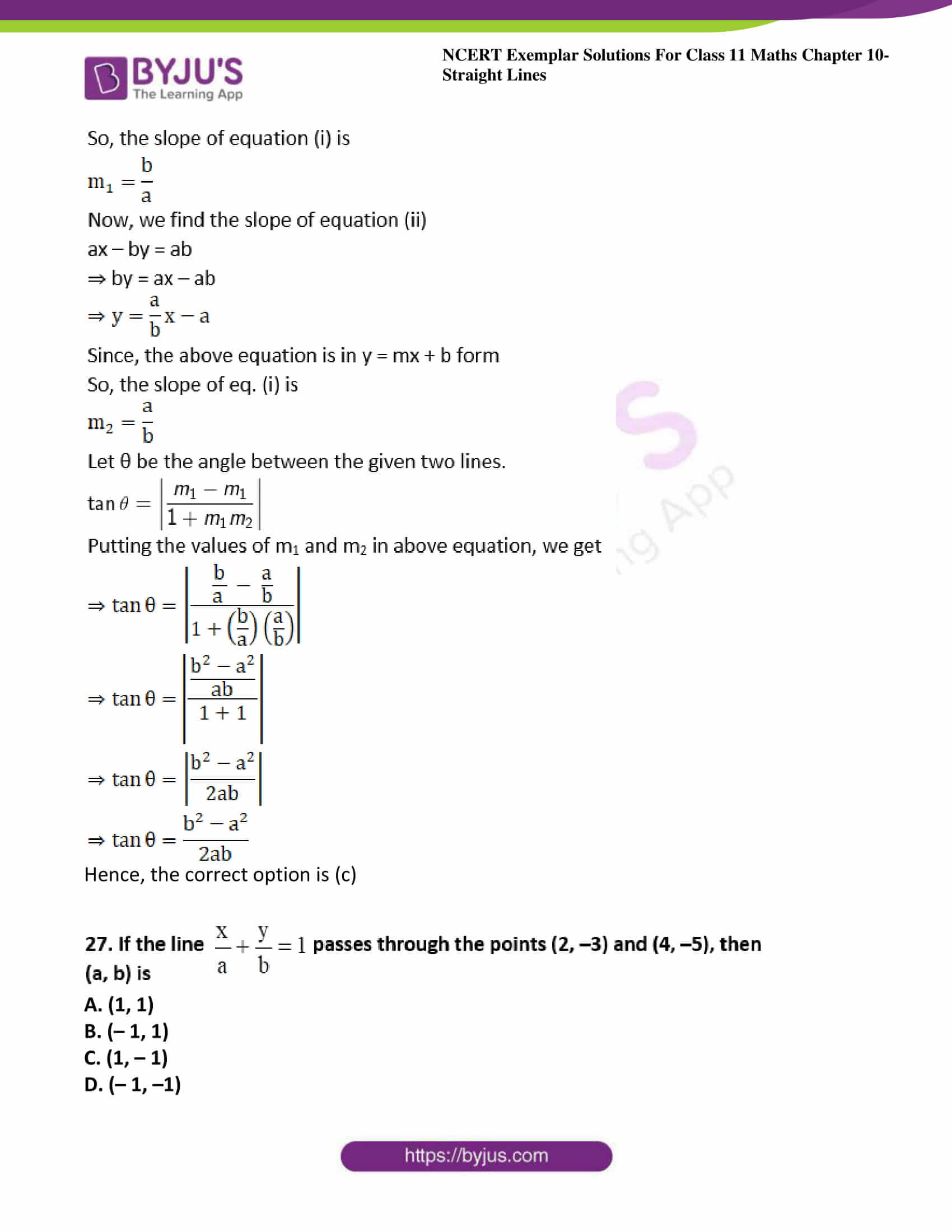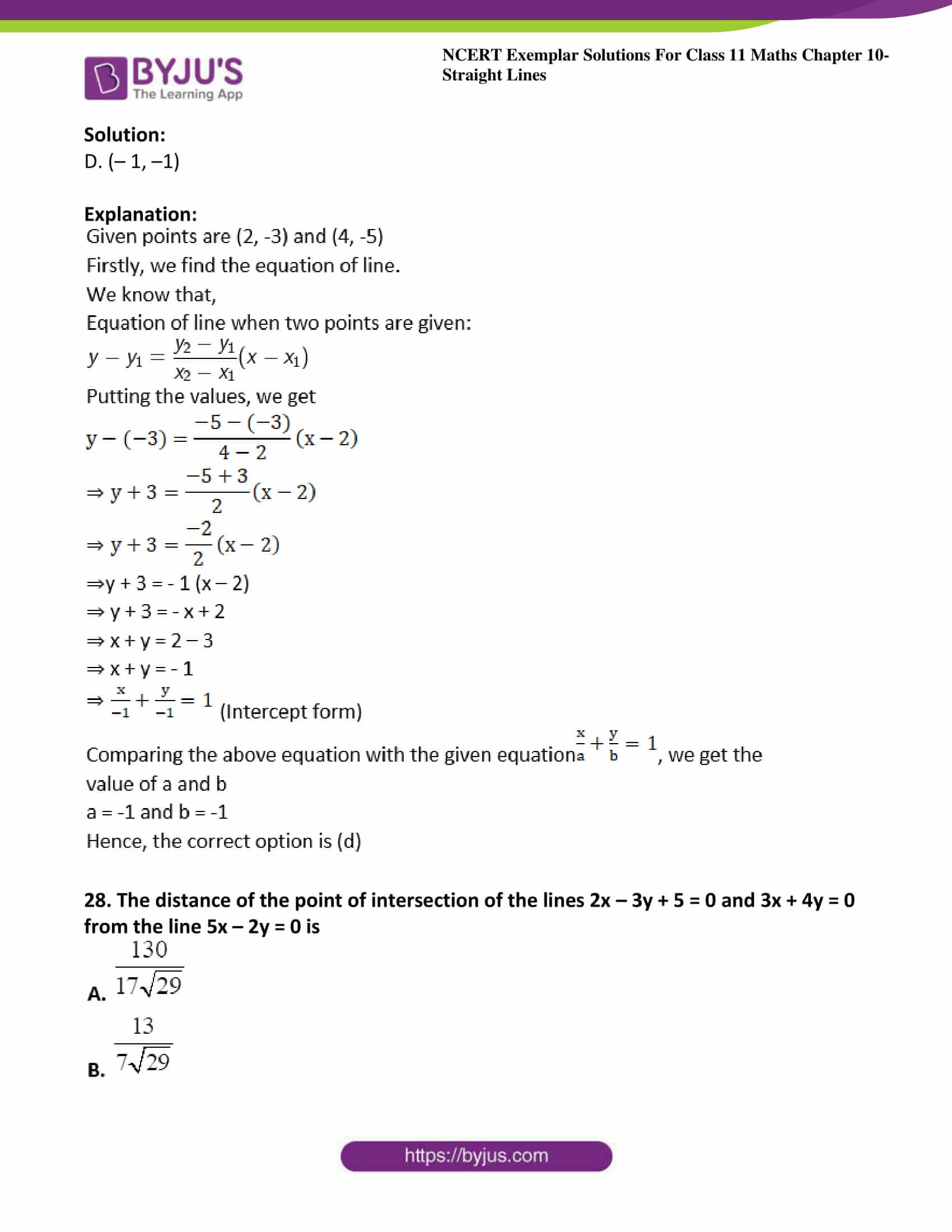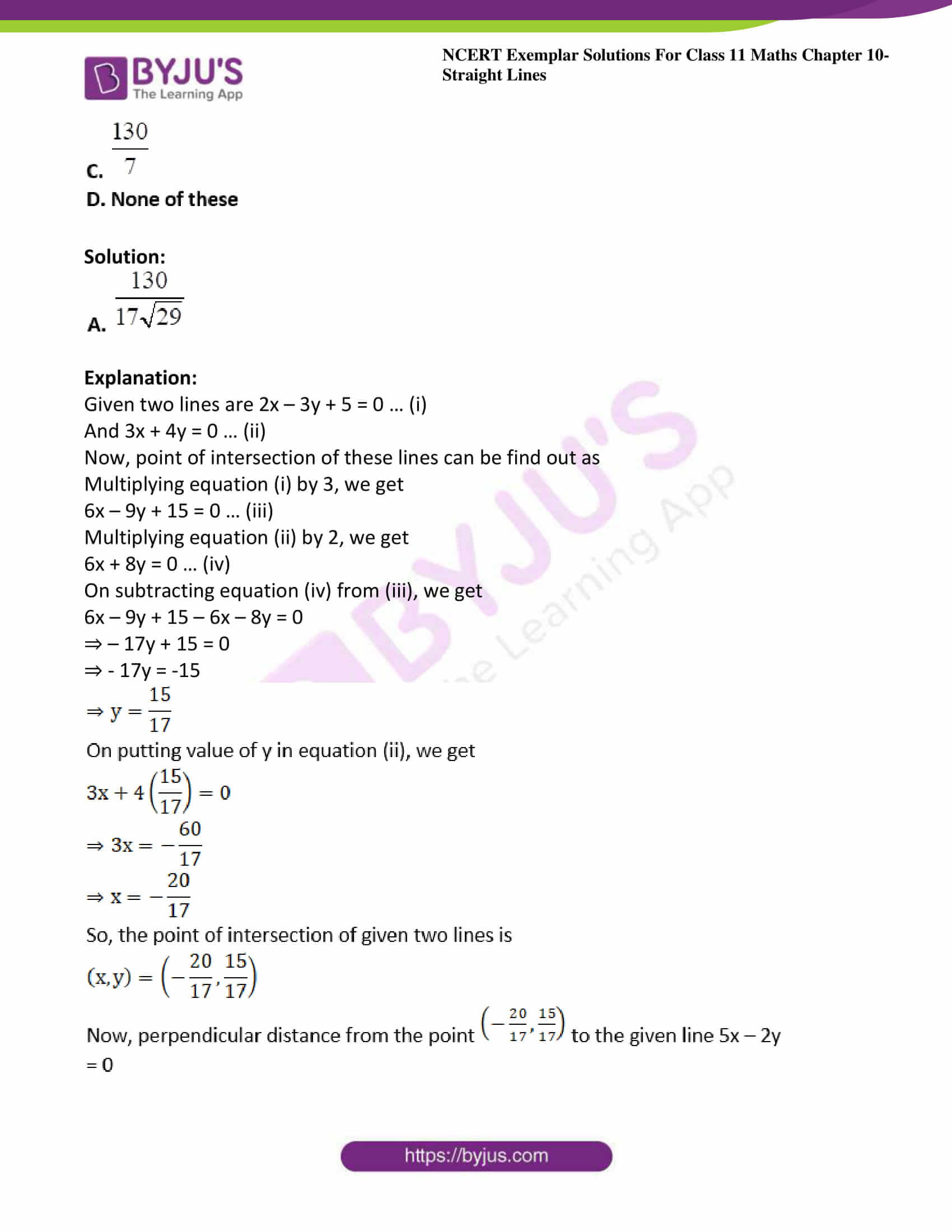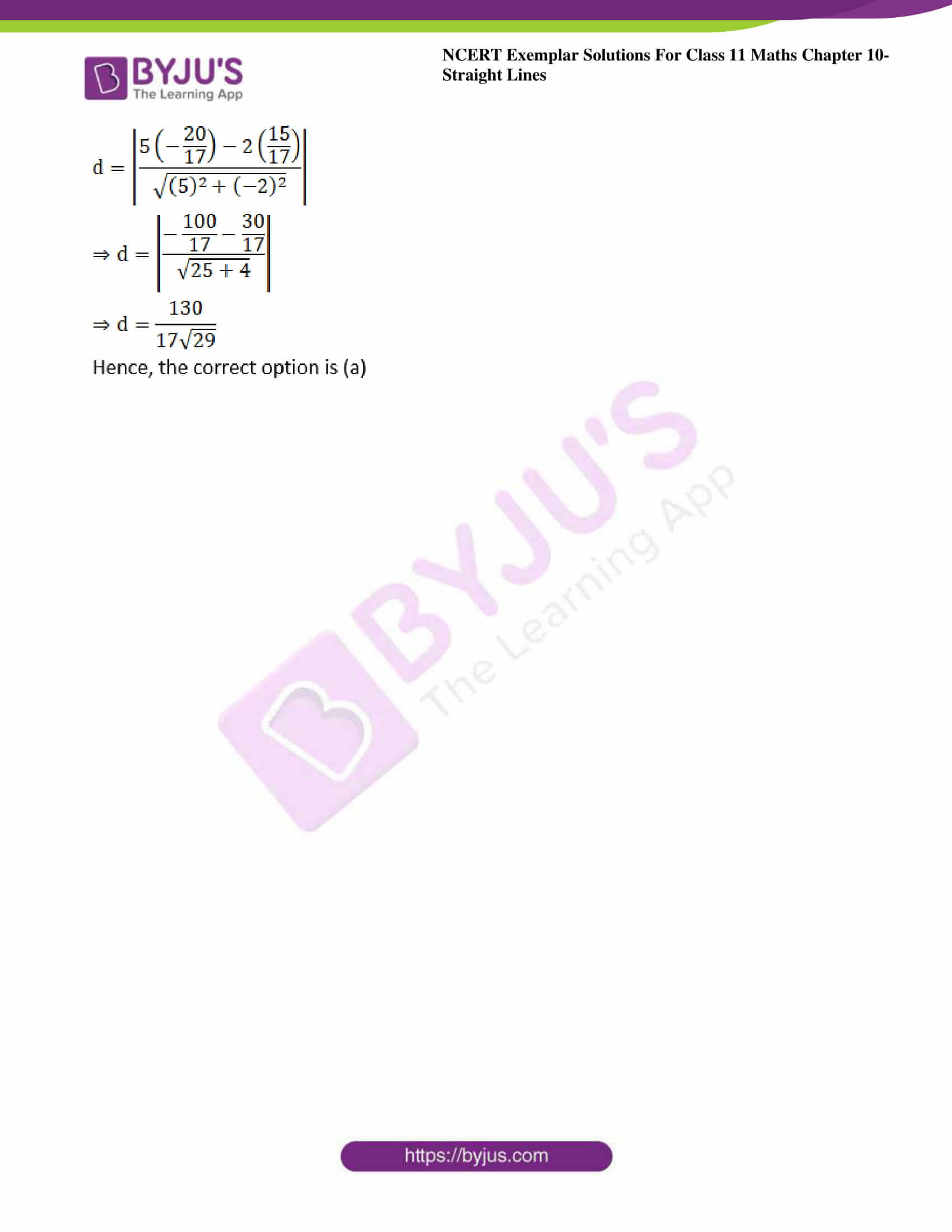### Access Answers to NCERT Exemplar Solutions for Class 11 Maths Chapter 10 – Straight Lines

Exercise Page No: 178

1. Find the equation of the straight line which passes through the point (1, – 2) and cuts off equal intercepts from axes.

Solution: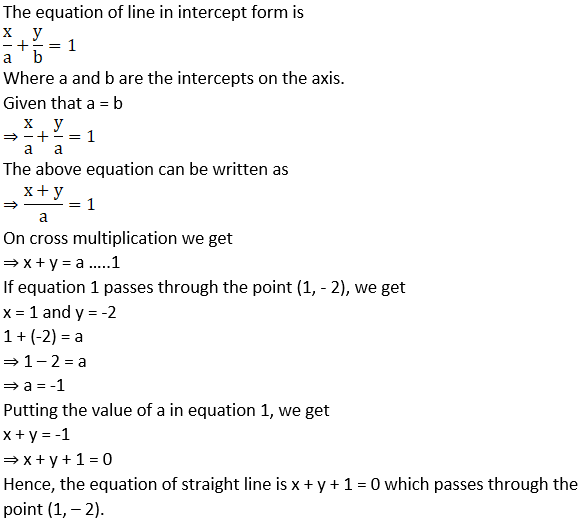2. Find the equation of the line passing through the point (5, 2) and perpendicular to the line joining the points (2, 3) and (3, – 1).

Solution:

Given points are A (5, 2), B (2, 3) and C (3, -1)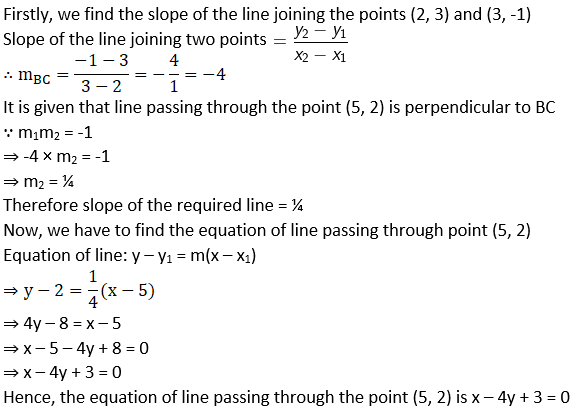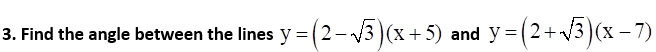Solution: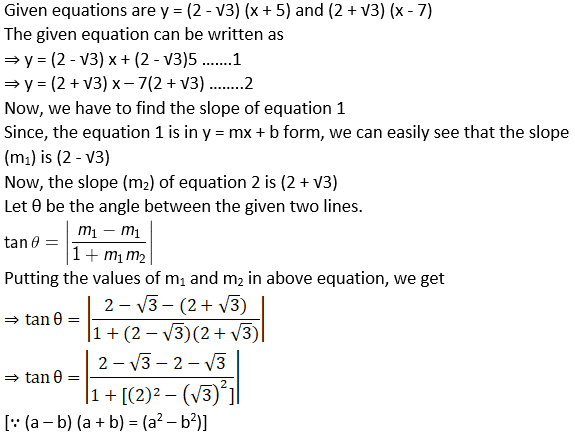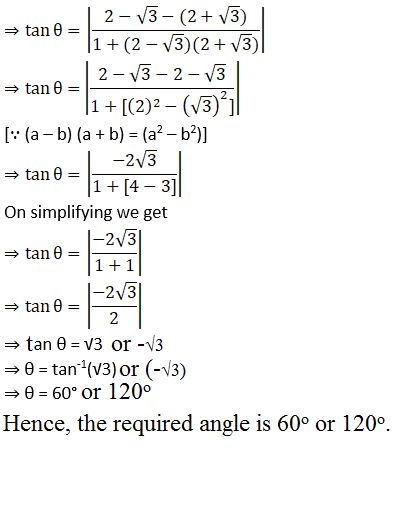4. Find the equation of the lines which passes through the point (3, 4) and cuts off intercepts from the coordinate axes such that their sum is 14.

Solution: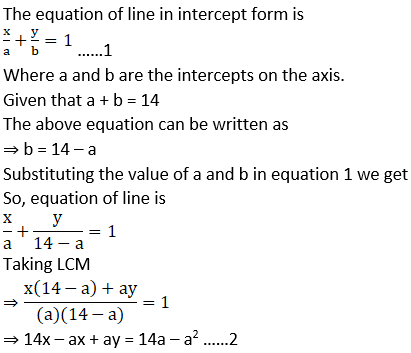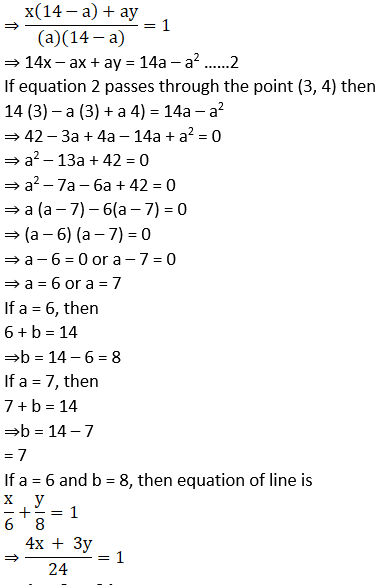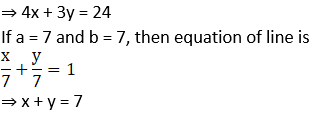5. Find the points on the line x + y = 4 which lie at a unit distance from the line 4x + 3y = 10.

Solution:

Let (x1, y1) be any point lying in the equation x+ y = 4

∴ x1 + y1 = 4 …..1

Distance of the point (x1, y1) from the equation 4x + 3y = 10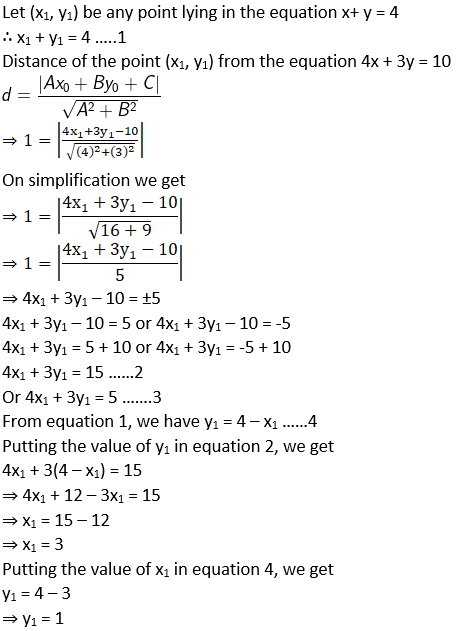Putting the value of y1 = 4 – x1 in equation 3, we get

4x1 + 3(4 – x1) = 5

⇒ 4x1 + 12 – 3x1 = 5

⇒ x1 = 5 – 12

⇒ x1 = – 7

Putting the value of x1 in equation 4, we get

y1 = 4 – (-7)

⇒ y1 = 4 + 7

⇒ y1 = 11

Hence, the required points on the given line are (3, 1) and (-7, 11)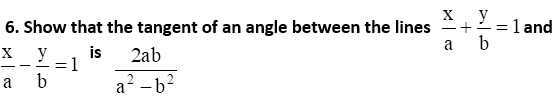Solution: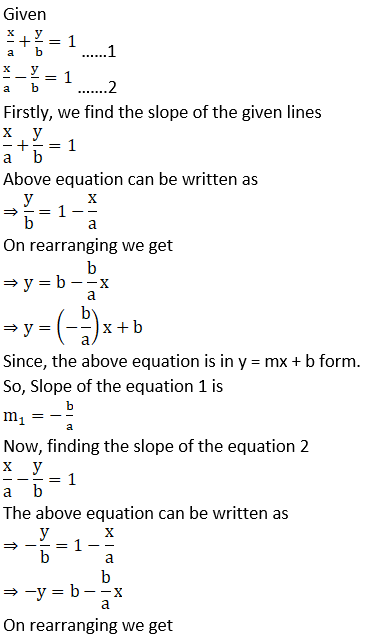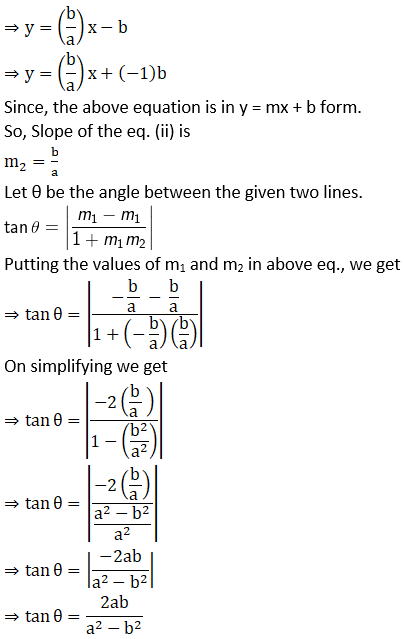Hence the proof.

7. Find the equation of lines passing through (1, 2) and making angle 30° with y-axis.

Solution: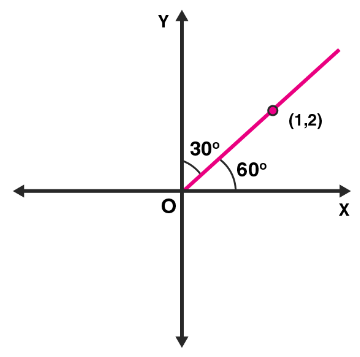Given that line passing through (1, 2) making an angle 30° with y – axis.

Angle made by the line with x – axis is (90° – 30°) = 60°

∴ Slope of the line, m = tan 60°

= √3

So, the equation of the line passing through the point (x1, y1) and having slope ‘m’ is

y – y1 = m (x – x1)

Here, (x1, y1) = (1, 2) and m = √3

⇒ y – 2 = √3(x – 1)

⇒ y – 2 = √3x – √3

⇒ y – √3x + √3 – 2 = 0

8. Find the equation of the line passing through the point of intersection of 2x + y = 5 and x + 3y + 8 = 0 and parallel to the line 3x + 4y = 7.

Solution:

Given lines are

2x + y = 5 ……1

x + 3y = -8 ……2

Firstly, we find the point of intersection of equation 1 and equation 2

Multiply the equation 2 by 2, we get

2x + 6y = -16 …….3

On subtracting equation 3 from 1, we get

2x + y – 2x – 6y = 5 – (-16)

On simplifying we get

⇒ -5y = 5 + 16

⇒ -5y = 21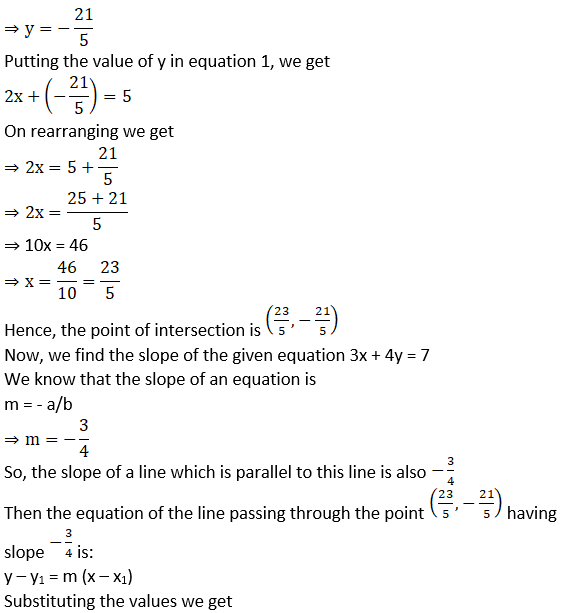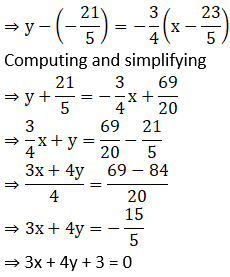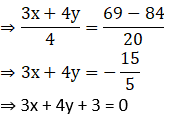9. For what values of a and b the intercepts cut off on the coordinate axes by the line ax + by + 8 = 0 are equal in length but opposite in signs to those cut off by the line 2x – 3y + 6 = 0 on the axes.

Solution: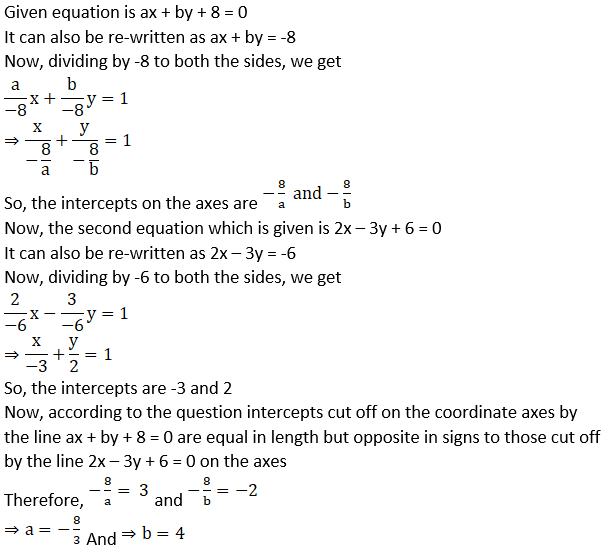10. If the intercept of a line between the coordinate axes is divided by the point (–5, 4) in the ratio 1:2, then find the equation of the line.

Solution: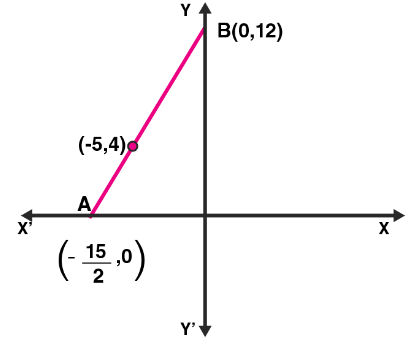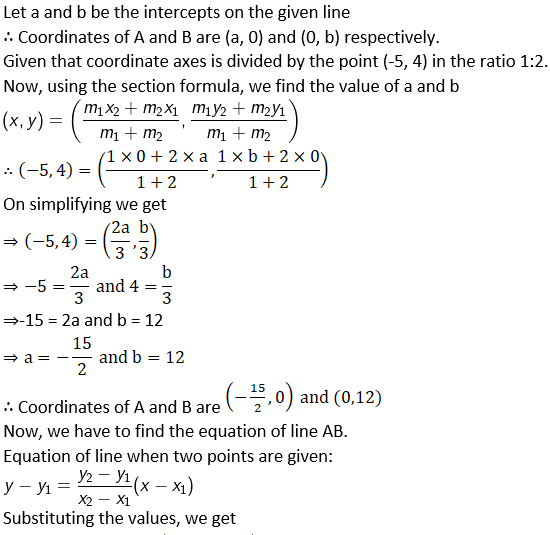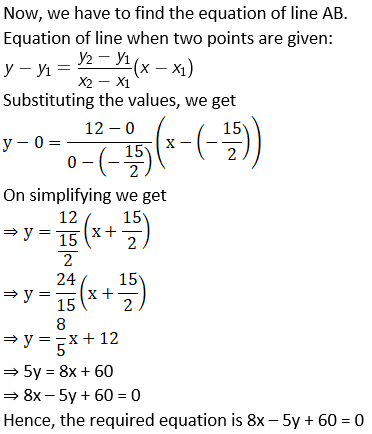11. Find the equation of a straight line on which length of perpendicular from the origin is four units and the line makes an angle of 120° with the positive direction of x-axis.

Solution: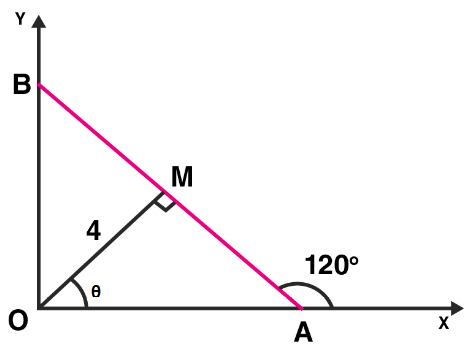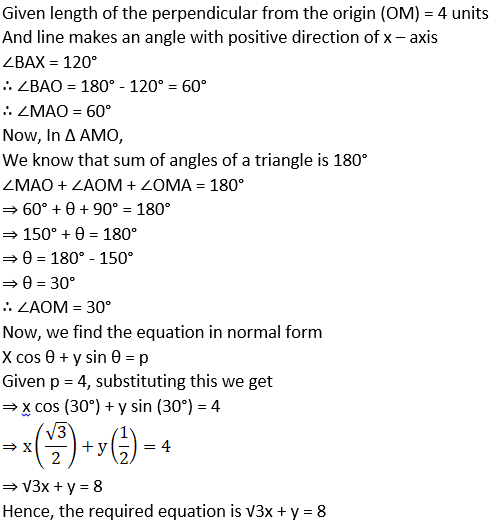12. Find the equation of one of the sides of an isosceles right angled triangle whose hypotenuse is given by 3x + 4y = 4 and the opposite vertex of the hypotenuse is (2, 2).

Solution: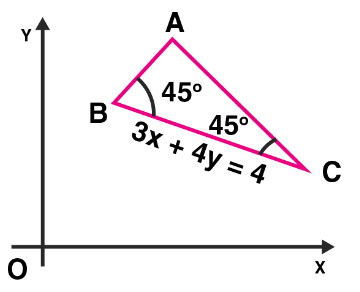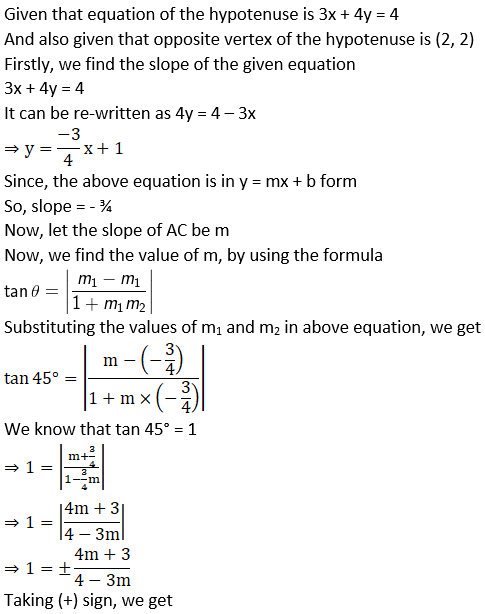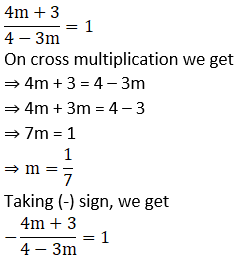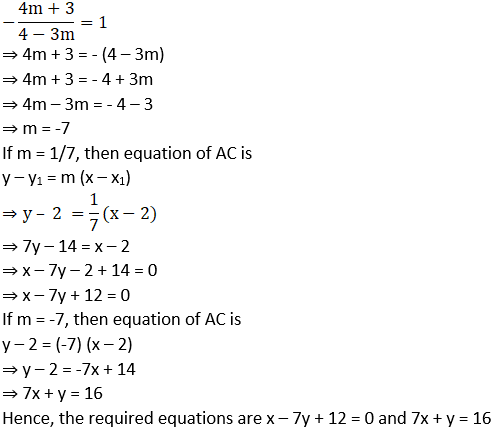13. If the equation of the base of an equilateral triangle is x + y = 2 and the vertex is (2, – 1), then find the length of the side of the triangle.

Solution: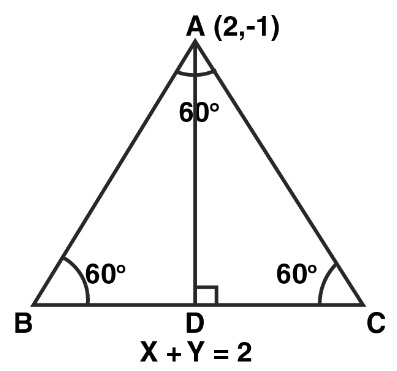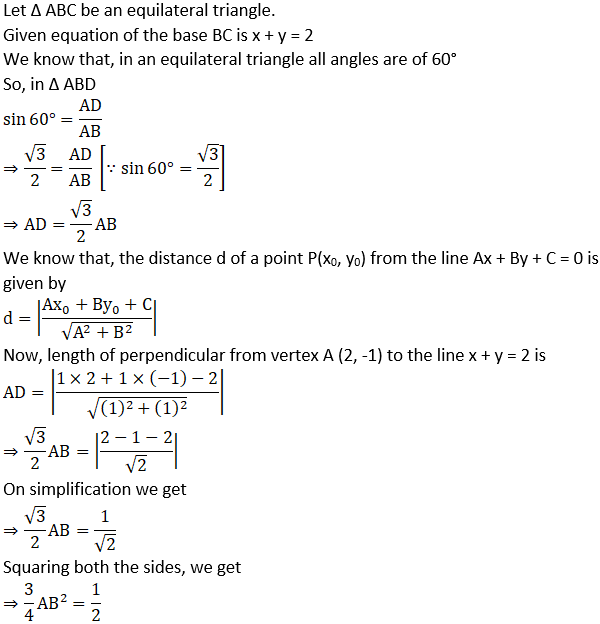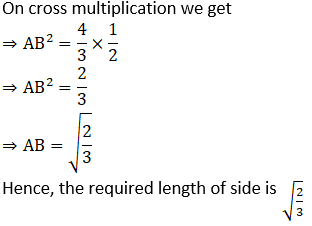14. A variable line passes through a fixed point P. The algebraic sum of the perpendiculars drawn from the points (2, 0), (0, 2) and (1, 1) on the line is zero. Find the coordinates of the point P.

Solution: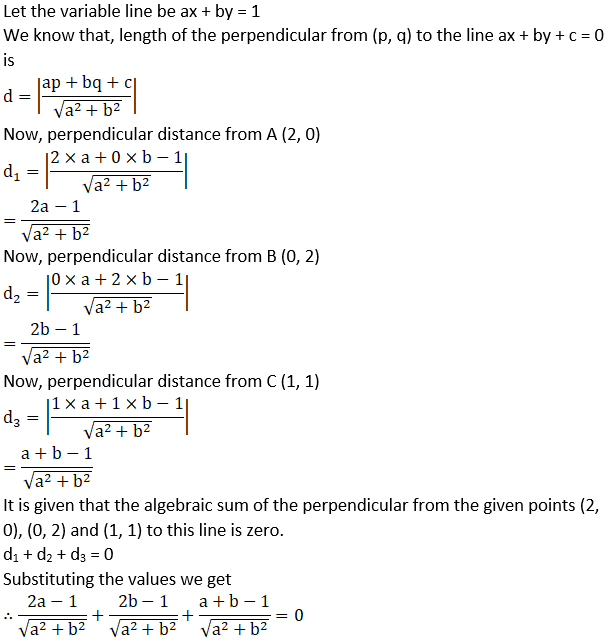⇒ 2a – 1 + 2b – 1 + a + b – 1 = 0

⇒ 3a + 3b – 3 = 0

⇒ a + b – 1 = 0

⇒ a + b = 1

So, the equation ax + by = 1 represents a family of straight lines passing through a fixed point.

Comparing the equation ax + by = 1 and a + b = 1, we get

x = 1 and y = 1

So, the coordinates of fixed point is (1, 1)

15. In what direction should a line be drawn through the point (1, 2) so that its point of intersection with the line x + y = 4 is at a distance √6/3 from the given point.

Solution: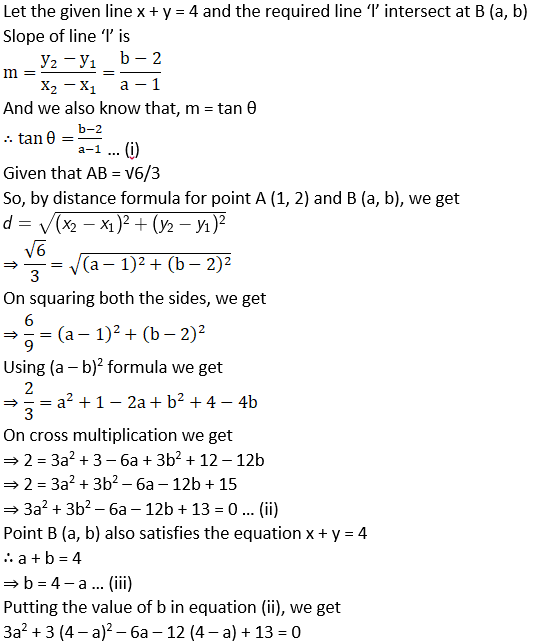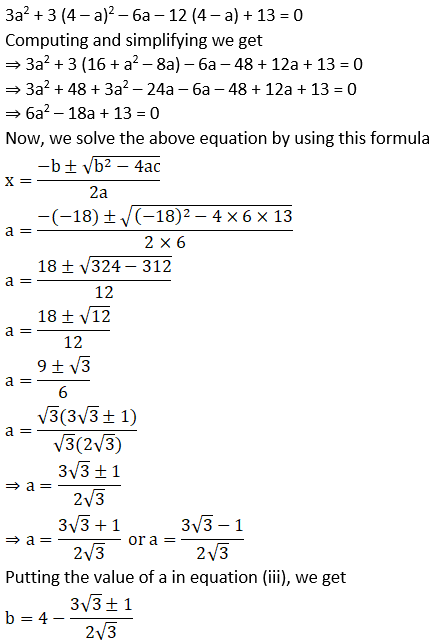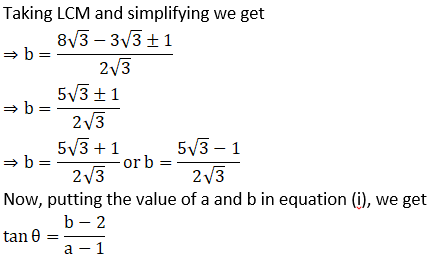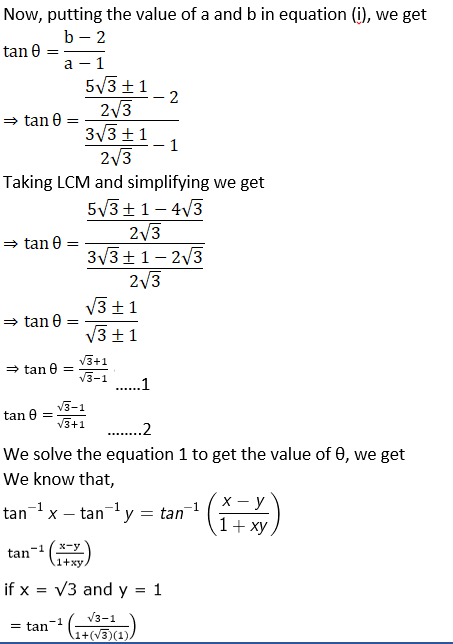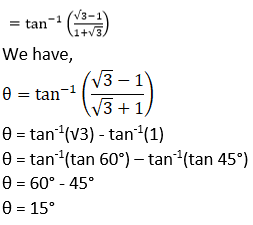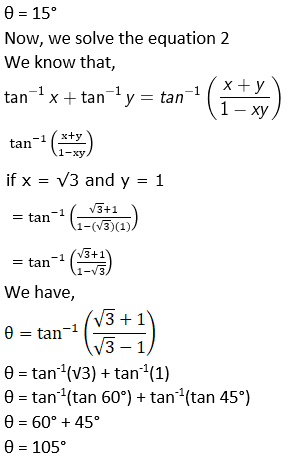16. A straight line moves so that the sum of the reciprocals of its intercepts made on axes is constant. Show that the line passes through a fixed point.

Solution: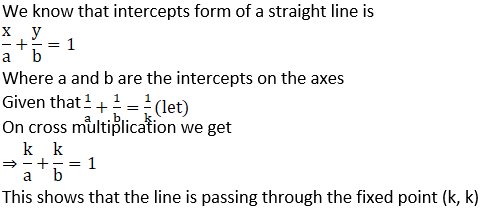17. Find the equation of the line which passes through the point (– 4, 3) and the portion of the line intercepted between the axes is divided internally in the ratio 5 : 3 by this point.

Solution: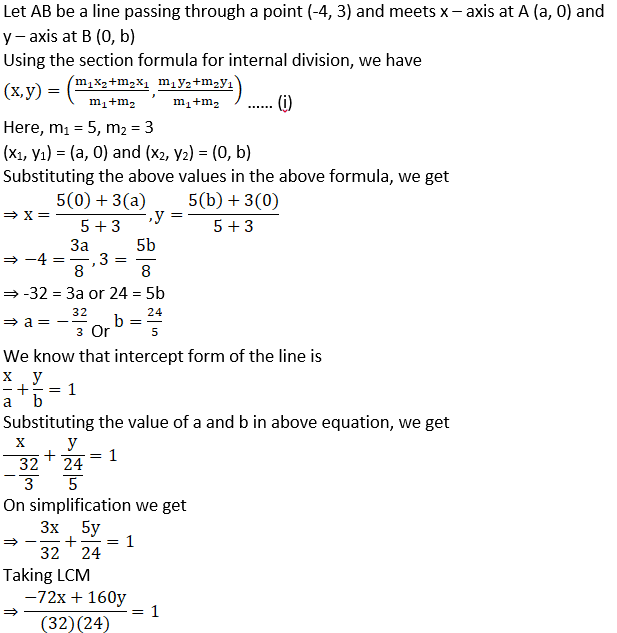On cross multiplication we get

⇒ -72x + 160y = 768

⇒ -36x + 80y = 384

⇒ 18x – 40y + 192 = 0

⇒ 9x – 20y + 96 = 0

Hence, the required equation is 9x – 20y + 96 = 0

18. Find the equations of the lines through the point of intersection of the lines x – y + 1 = 0 and 2x – 3y + 5 = 0 and whose distance from the point (3, 2) is 7/5.

Solution: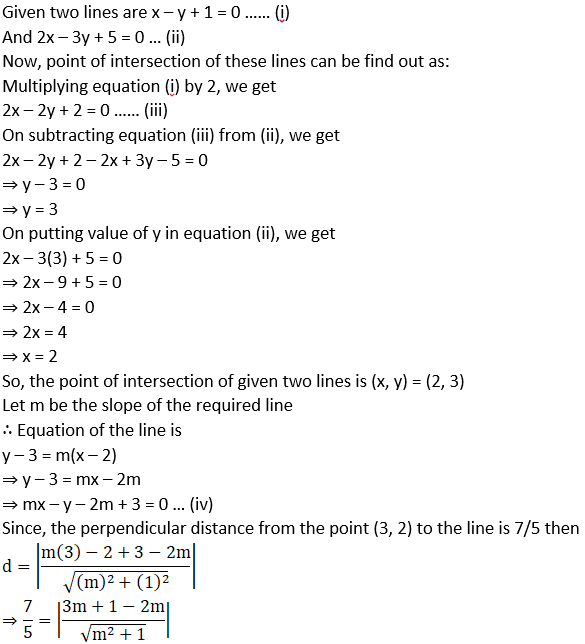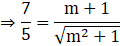Squaring both the sides, we get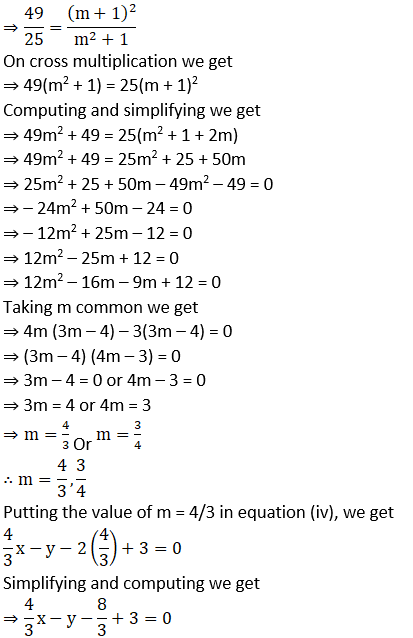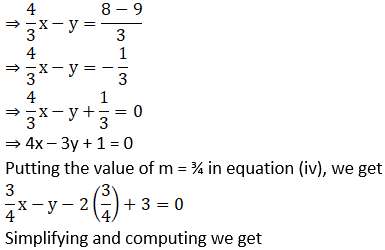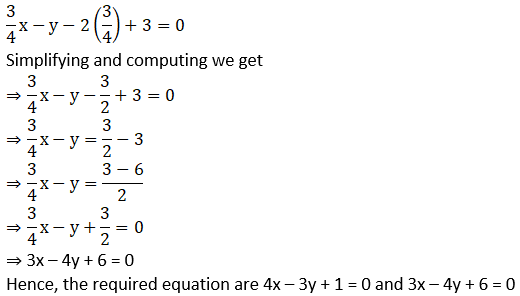19. If the sum of the distances of a moving point in a plane from the axes is 1, then find the locus of the point.

Solution: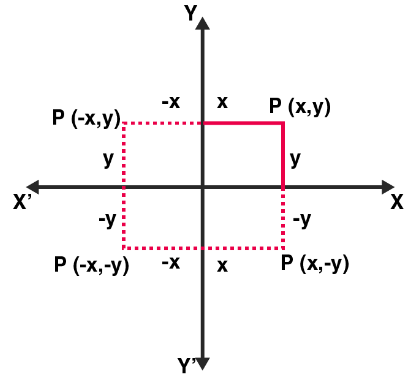Let the coordinates of a moving point P be (a, b)

Given that the sum of the distance from the axes to the point is always 1

∴ |x| + |y| = 1

⇒ ±x ± y = 1

⇒ – x – y = 1, x + y = 1, -x + y = 1 and x – y = 1

Hence, these equations give us the locus of point P, which is a square.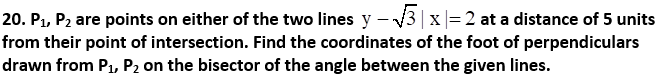Solution:

Given lines are y – √3|x| = 2

If x ≥ 0, then

y – √3x = 2 ….. (i)

If x < 0, then

y + √3x = 2 ….. (ii)

On adding equation (i) and (ii), we get

y – √3x + y + √3x = 2 + 2

⇒ 2y = 4

⇒ y = 2

Substituting the value of y = 2 in equation (ii), we get

2 + √3x = 2

⇒ √3x = 2 – 2

⇒ x = 0

∴ Point of intersection of given lines is (0, 2)

Now, we find the slopes of given lines.

Slope of equation (i) is

y = √3x + 2

Comparing the above equation with y = mx + b, we get

m = √3

And we know that, m = tan θ

∴ Tan θ = √3

⇒ θ = 60° [∵ tan 60° = √3]

Slope of equation (ii) is

y = – √3x + 2

Comparing the above equation with y = mx + b, we get

m = -√3

And we know that, m = tan θ

∴ Tan θ = -√3

⇒ θ = (180° – 60°)

⇒ θ = 120°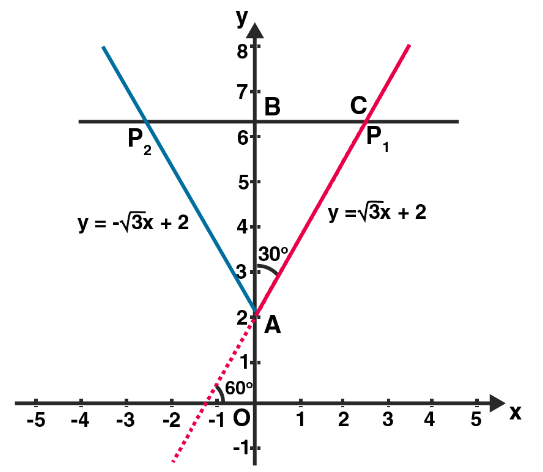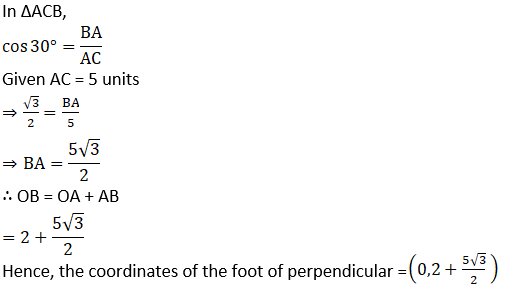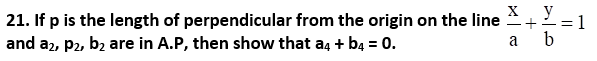Solution: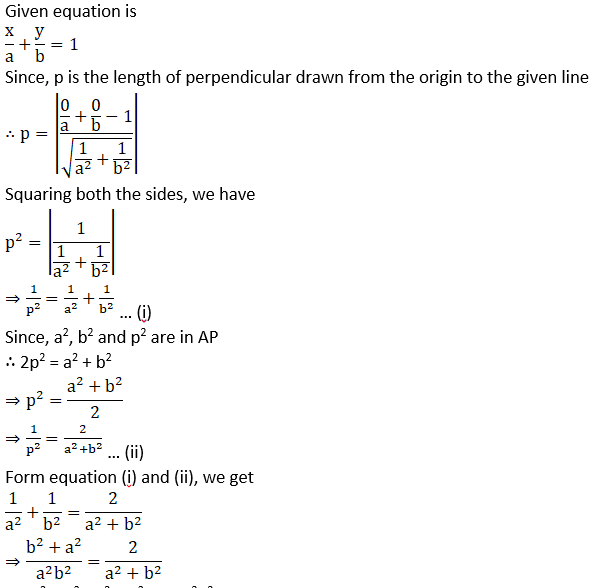⇒ (a2 + b2) (a2 + b2) = 2(a2b2)

⇒ a4 + b4 + a2b2 + a2b2 = 2a2b2

⇒ a4 + b4 = 0

Hence Proved

Objective Type Questions

22. A line cutting off intercept – 3 from the y-axis and the tangent at angle to the x-axis is 3/5, its equation is
A. 5y – 3x + 15 = 0

B. 3y – 5x + 15 = 0
C. 5y – 3x – 15 = 0
D. None of these

Solution:

A. 5y – 3x + 15 = 0

Explanation: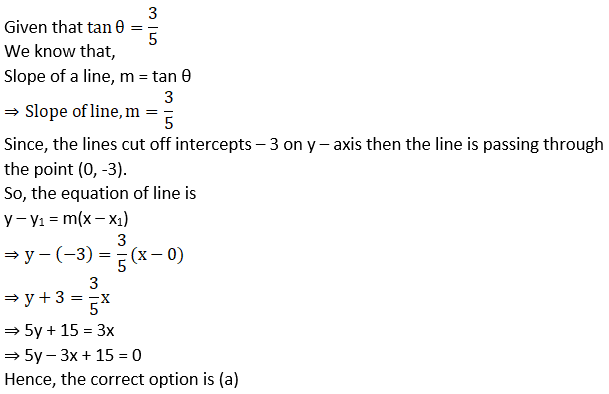23. Slope of a line which cuts off intercepts of equal lengths on the axes is
A. – 1
B. – 0
C. 2
D. √3

Solution:

A. – 1

Explanation: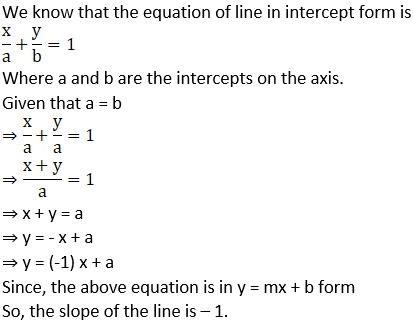24. The equation of the straight line passing through the point (3, 2) and perpendicular to the line y = x is
A. x – y = 5
B. x + y = 5
C. x + y = 1
D. x – y = 1

Solution:

B. x + y = 5

Explanation:

Given that straight line passing through the point (3, 2)

And perpendicular to the line y = x

Let the equation of line ‘L’ is

y – y1 = m(x – x1)

Since, L is passing through the point (3, 2)

∴ y – 2 = m(x – 3) … (i)

Now, given eq. is y = x

Since, the above equation is in y = mx + b form

So, the slope of this equation is 1

It is also given that line L and y = x are perpendicular to each other.

We know that, when two lines are perpendicular, then

m1 × m2 = -1

∴ m × 1 = -1

⇒ m = -1

Putting the value of m in equation (i), we get

y – 2 = (-1) (x – 3)

⇒ y – 2 = -x + 3

⇒ x + y = 3 + 2

⇒ x + y = 5

Hence, the correct option is (b)

25. The equation of the line passing through the point (1, 2) and perpendicular to the line x + y + 1 = 0 is
A. y – x + 1 = 0
B. y – x – 1 = 0
C. y – x + 2 = 0
D. y – x – 2 = 0

Solution:

B. y – x – 1 = 0

Explanation:

Given that line passing through the point (1, 2)

And perpendicular to the line x + y + 1 = 0

Let the equation of line ‘L’ is

x – y + k = 0 … (i)

Since, L is passing through the point (1, 2)

∴ 1 – 2 + k = 0

⇒ k = 1

Putting the value of k in equation (i), we get

x – y + 1 = 0

Or y – x – 1 = 0

Hence, the correct option is (b)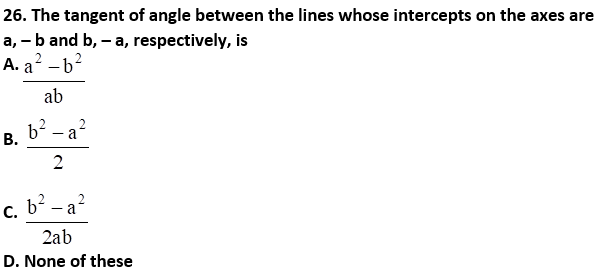Solution: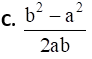Explanation: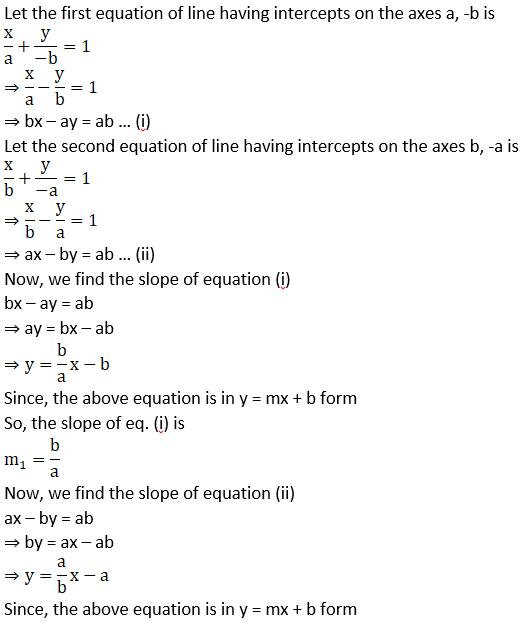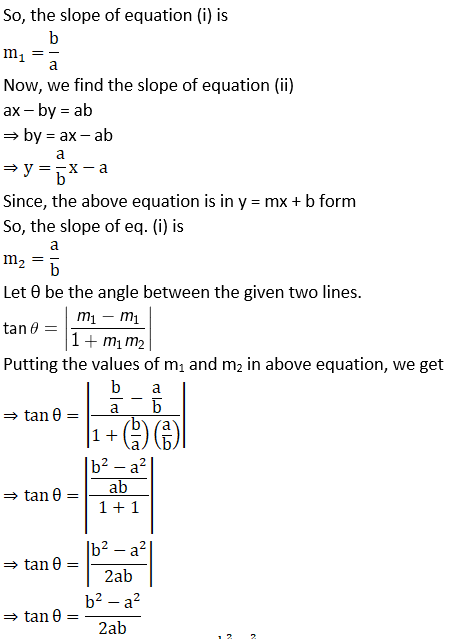Hence, the correct option is (c)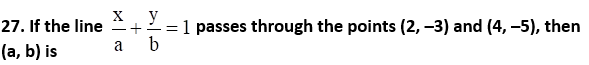A. (1, 1)
B. (– 1, 1)
C. (1, – 1)
D. (– 1, –1)

Solution:

D. (– 1, –1)

Explanation: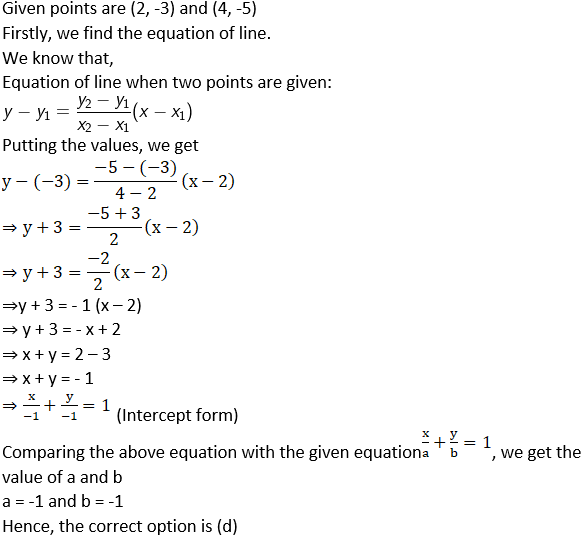28. The distance of the point of intersection of the lines 2x – 3y + 5 = 0 and 3x + 4y = 0 from the line 5x – 2y = 0 is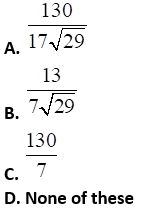Solution:Explanation:

Given two lines are 2x – 3y + 5 = 0 … (i)

And 3x + 4y = 0 … (ii)

Now, the point of intersection of these lines can be find out as

Multiplying equation (i) by 3, we get

6x – 9y + 15 = 0 … (iii)

Multiplying equation (ii) by 2, we get

6x + 8y = 0 … (iv)

On subtracting equation (iv) from (iii), we get

6x – 9y + 15 – 6x – 8y = 0

⇒ – 17y + 15 = 0

⇒ – 17y = -15